### 一、题目描述

输入：m = 2, n = 3, k = 1



输入：m = 3, n = 1, k = 0



• 1 <= n,m <= 100
• 0 <= k <= 20

### 二、题目解析

• 模拟：模拟题目的运行。
• 规律：尝试总结出题目的一般规律和特点。
• 匹配：找到符合这些特点的数据结构与算法。
• 边界：考虑特殊情况。

#### 1、模拟

• 避免少统计：遍历所有能移动的格子
• 避免重复统计：每次统计完一个格子，将其移除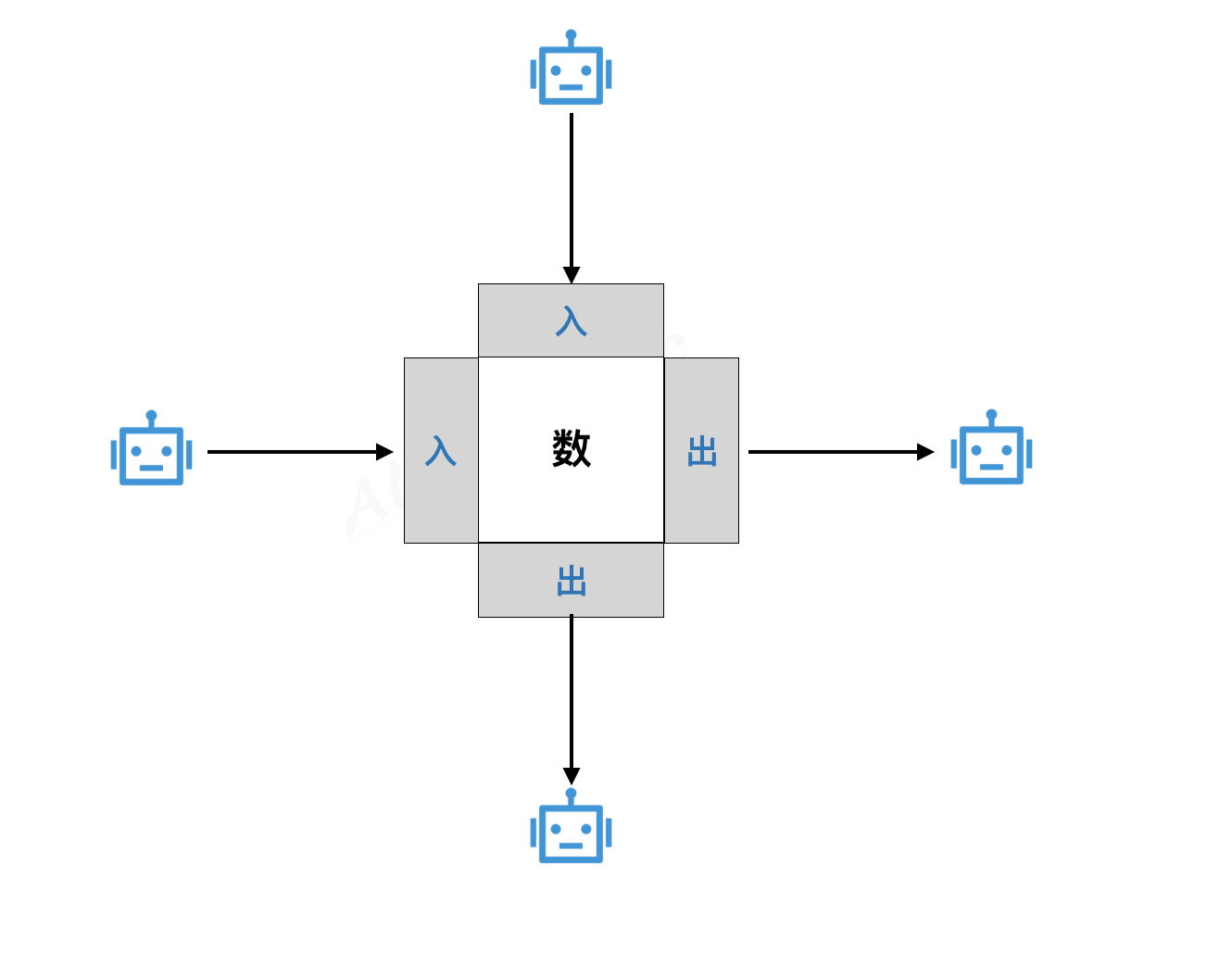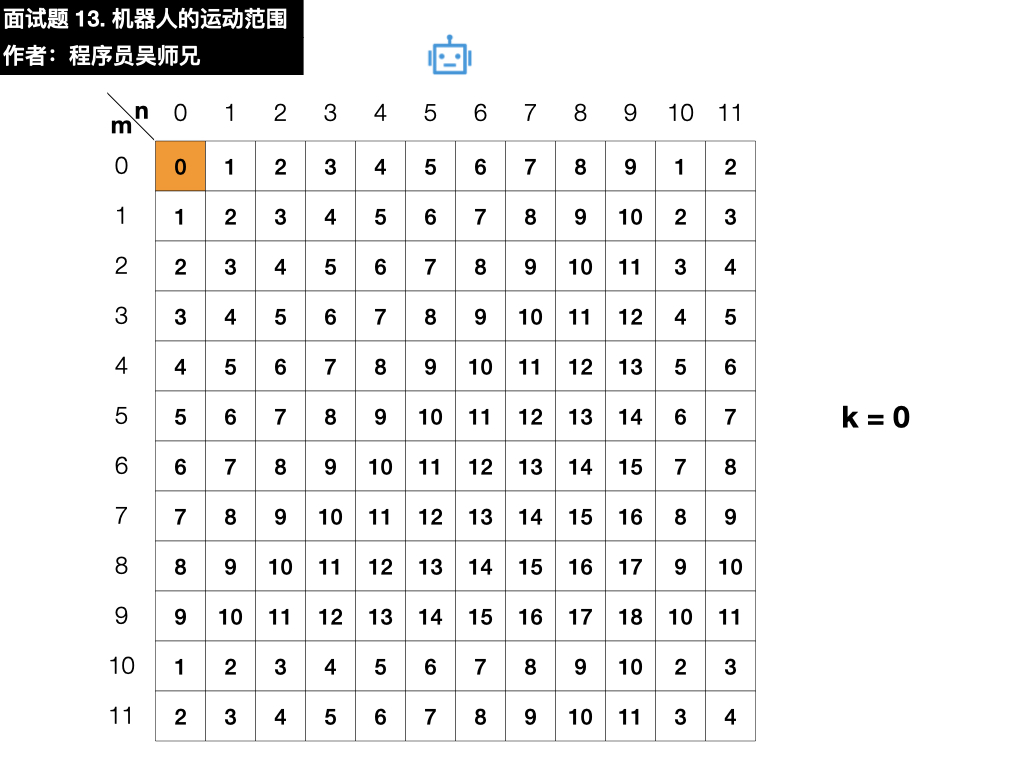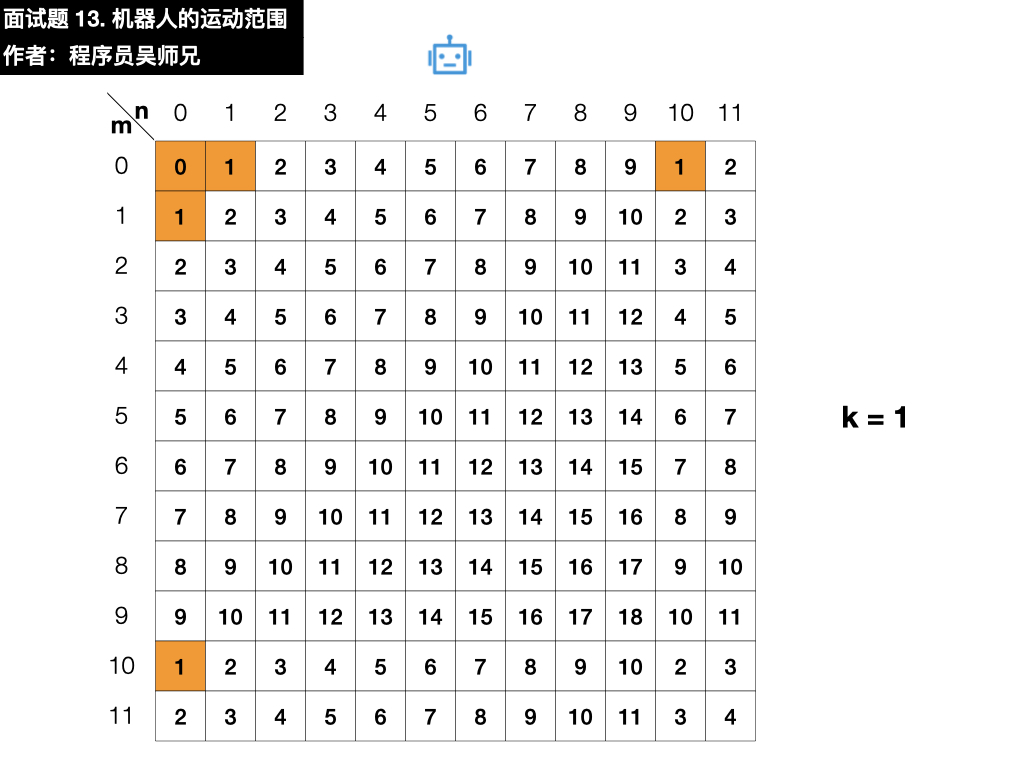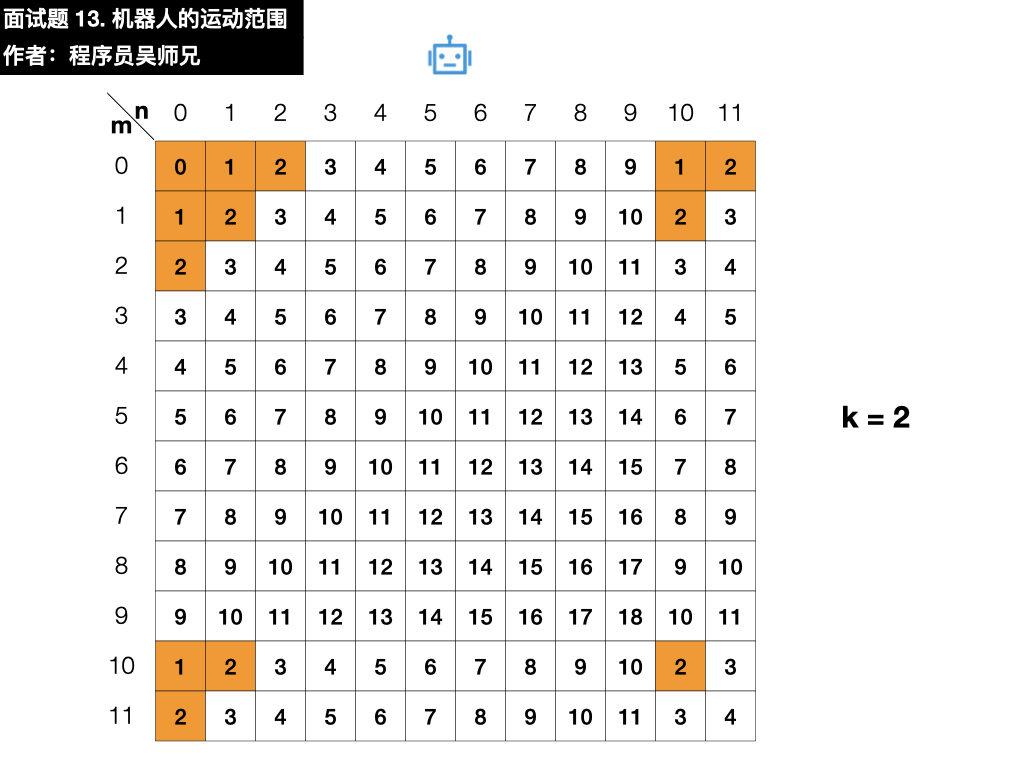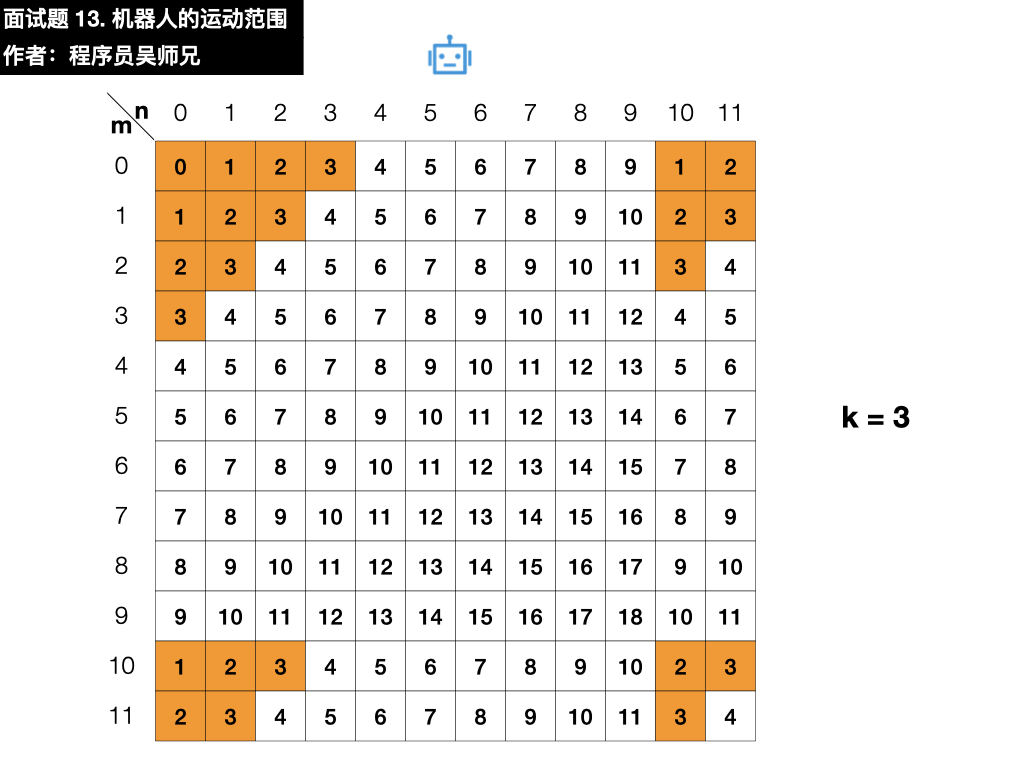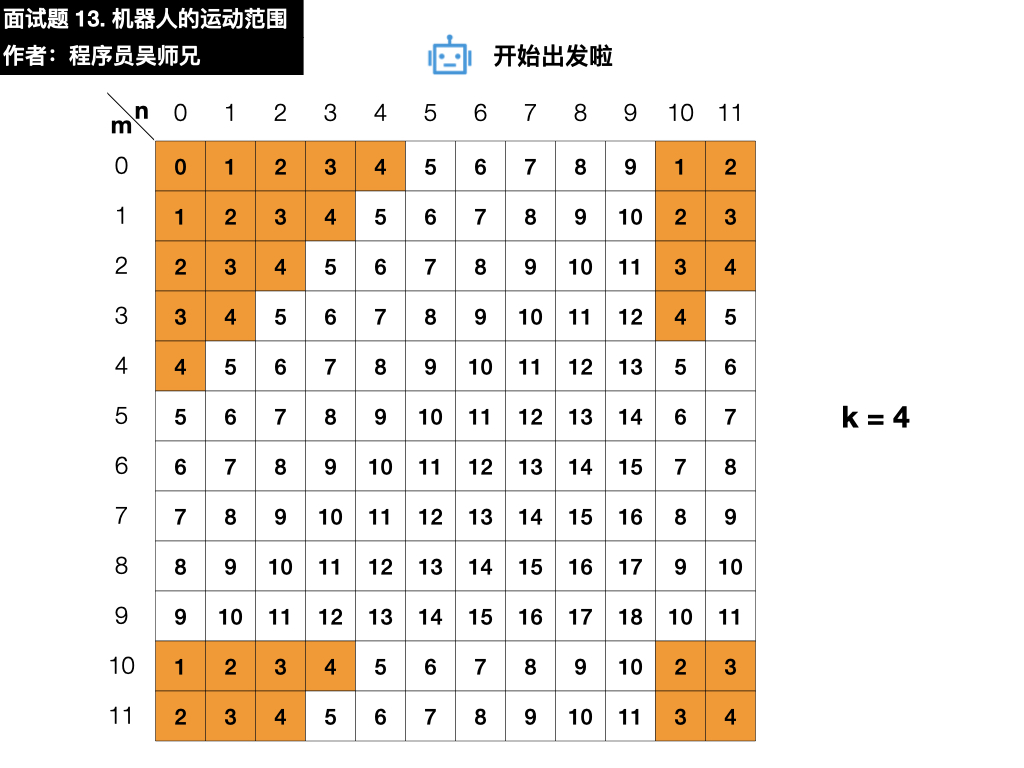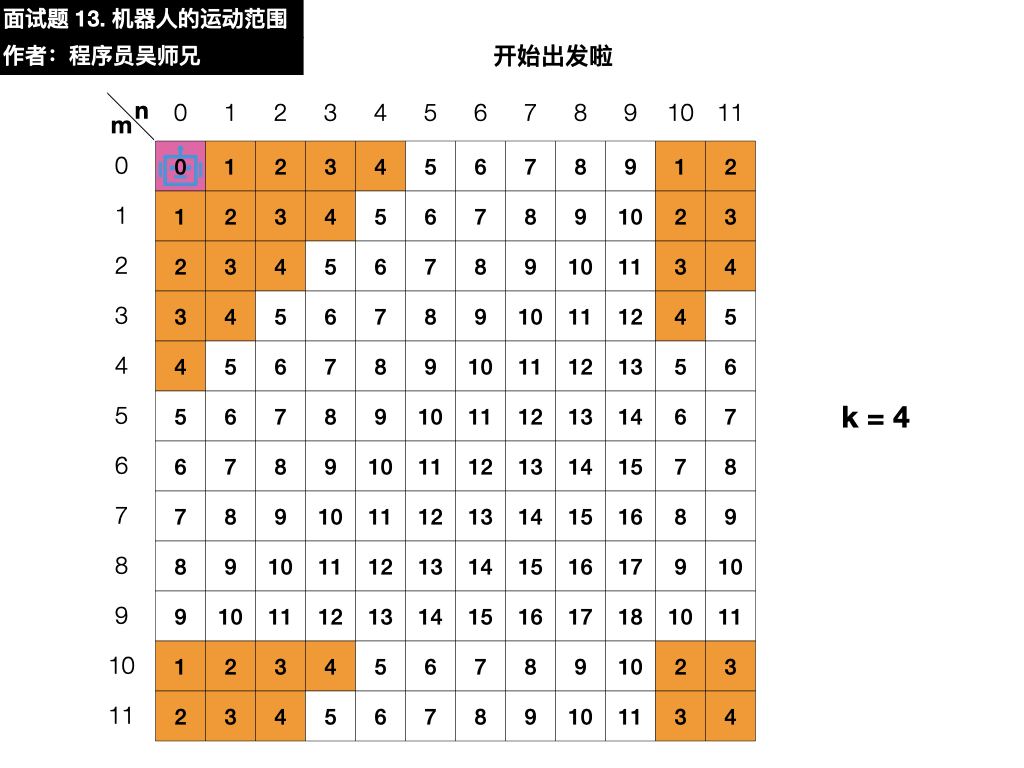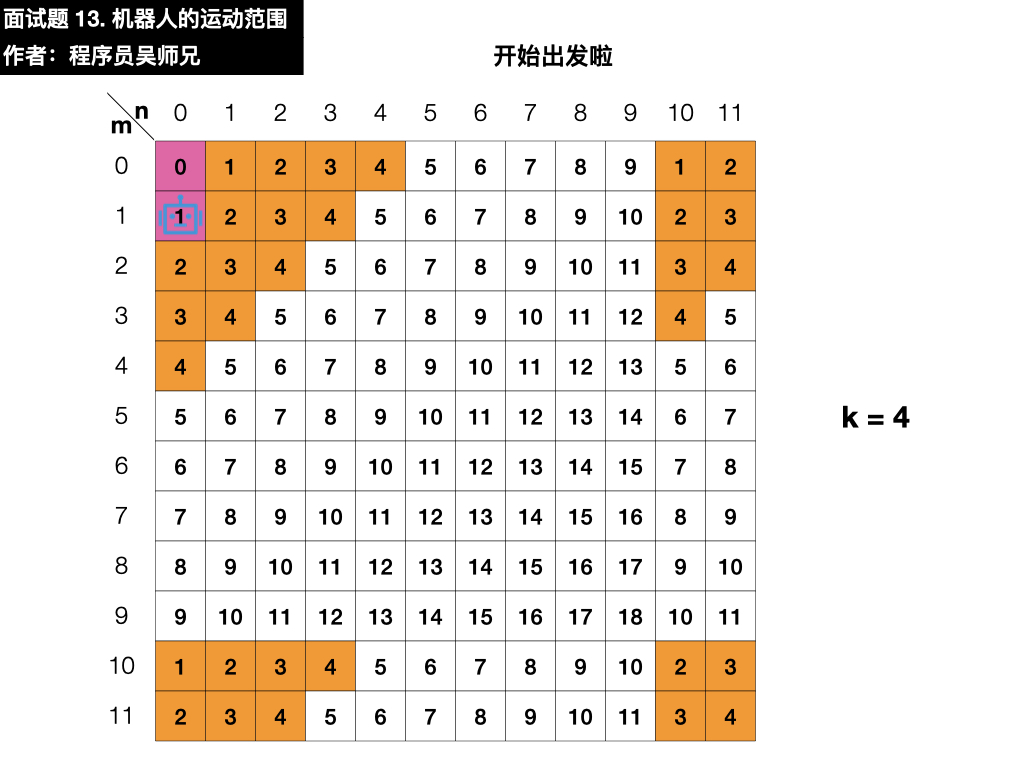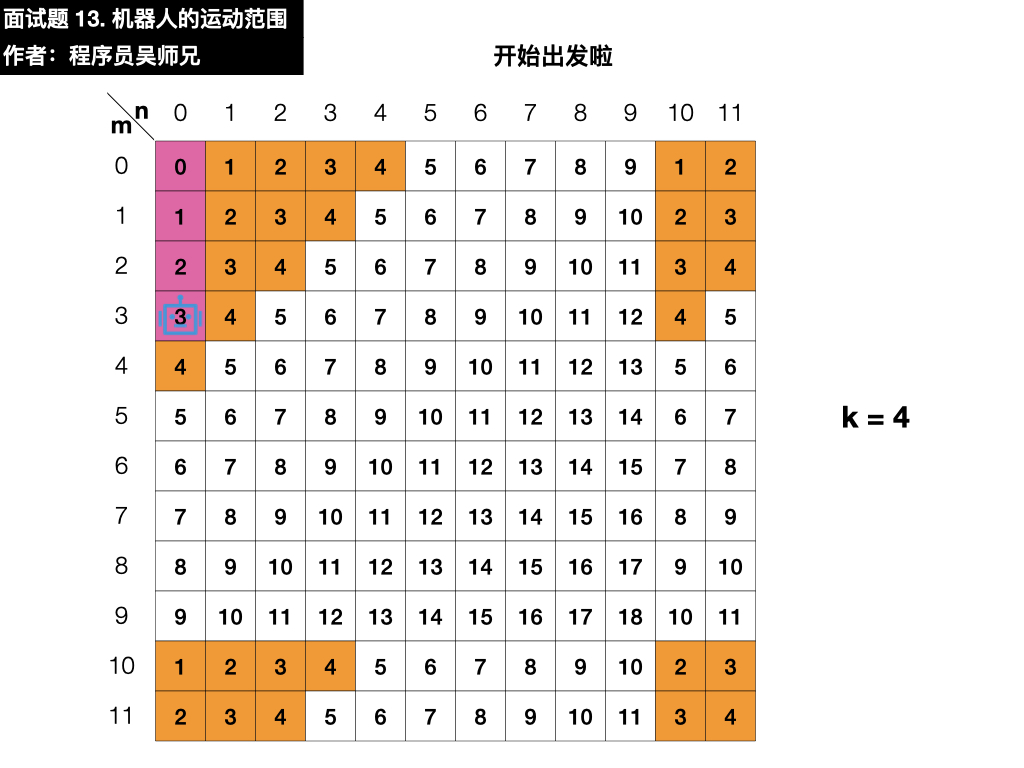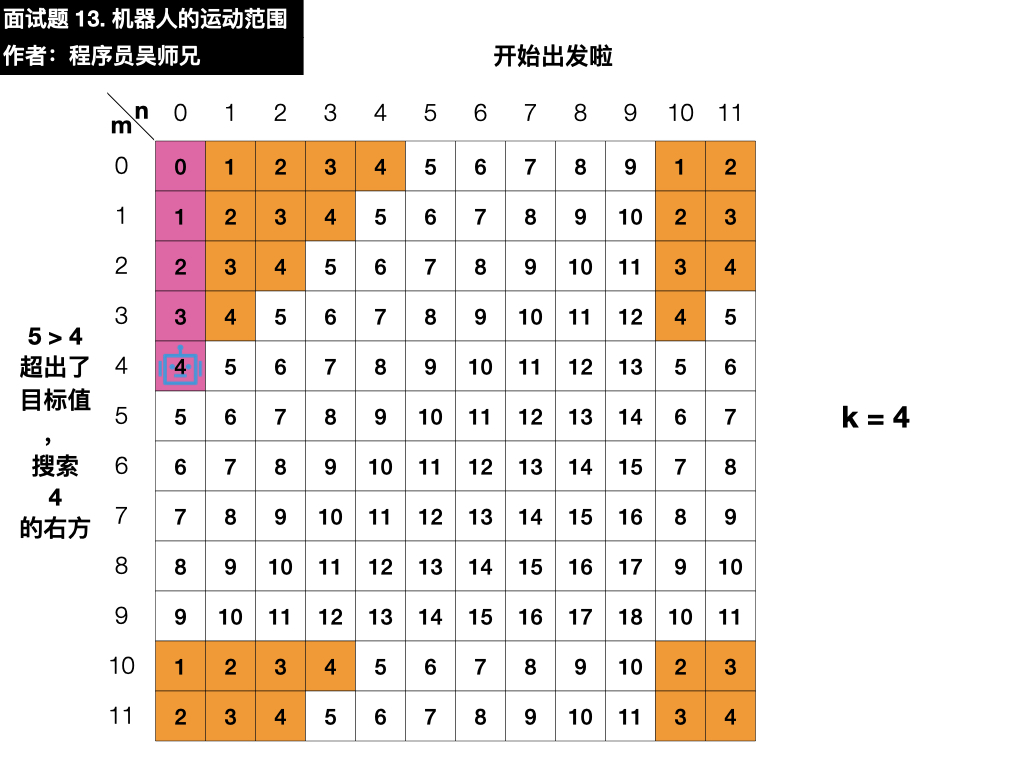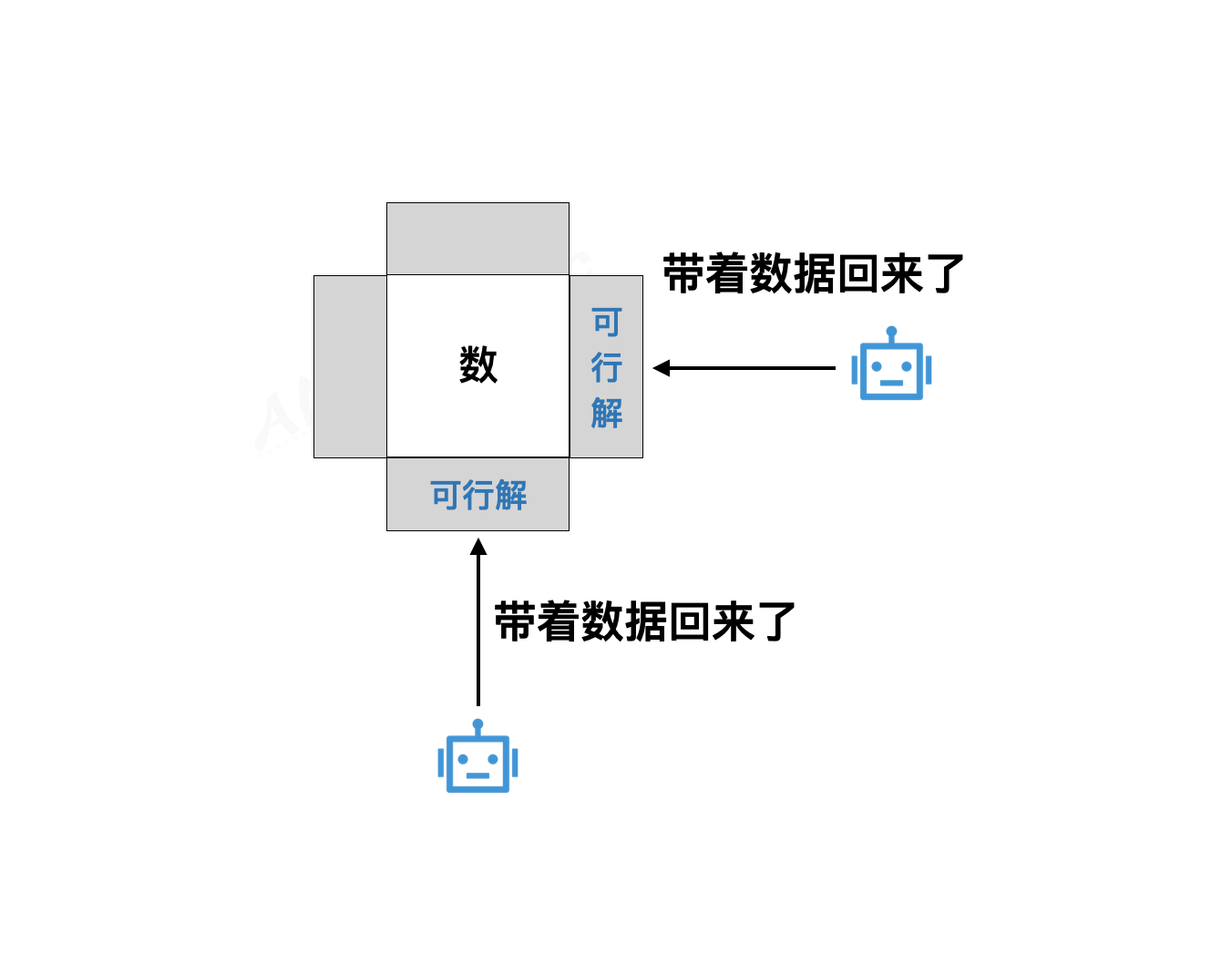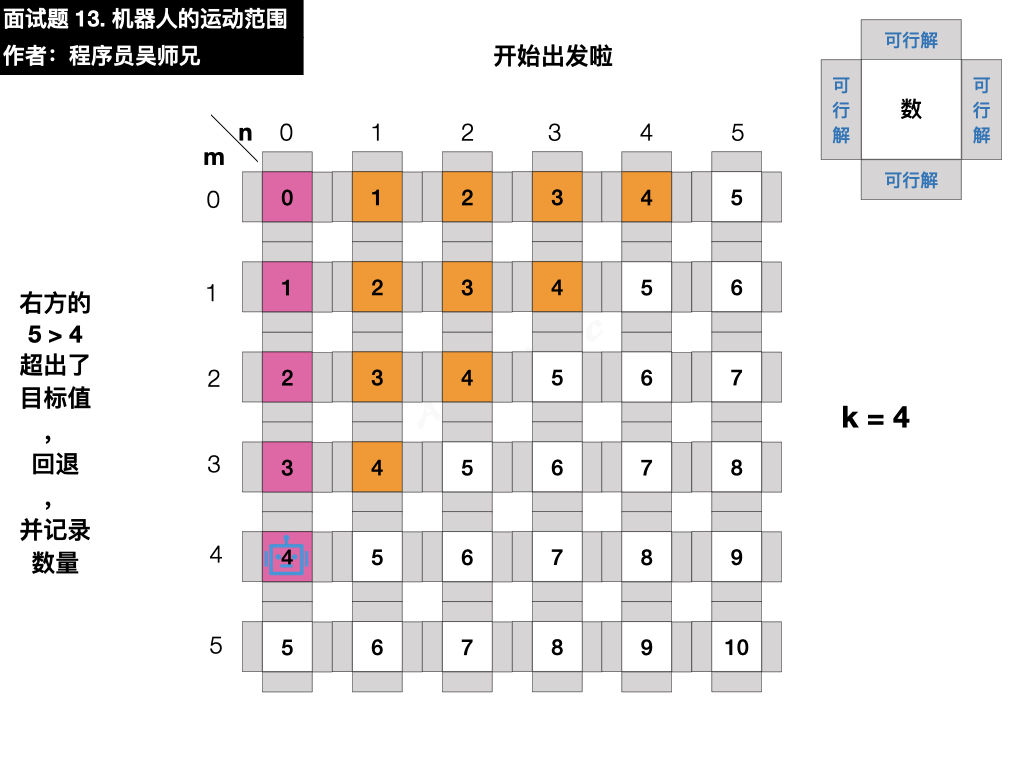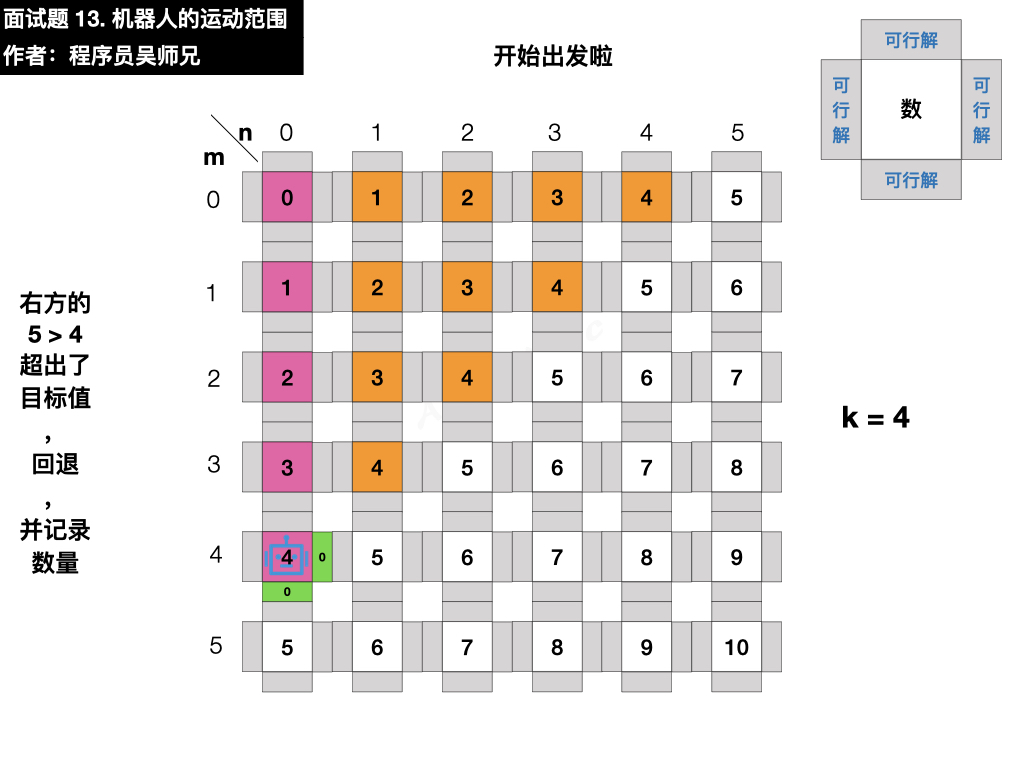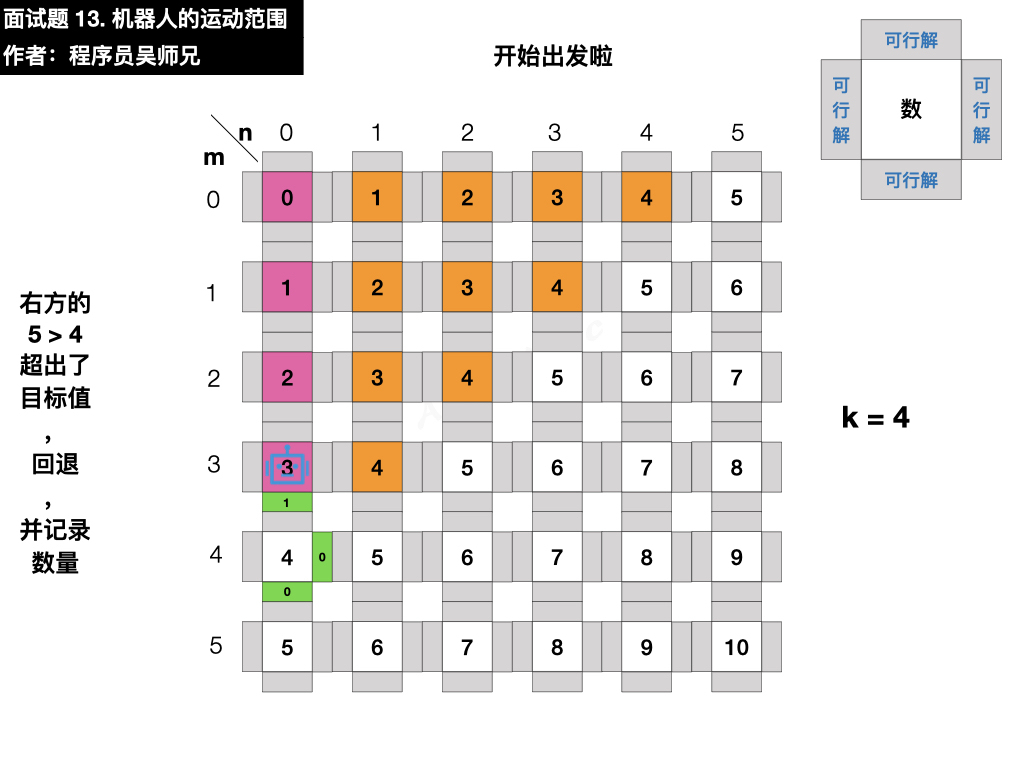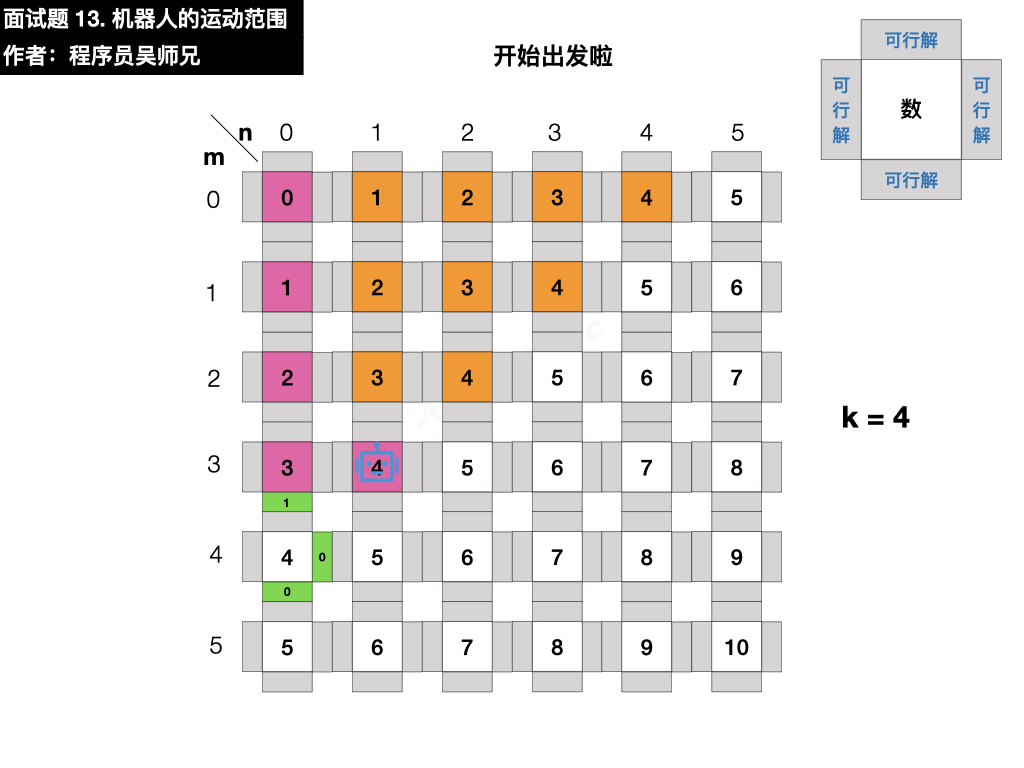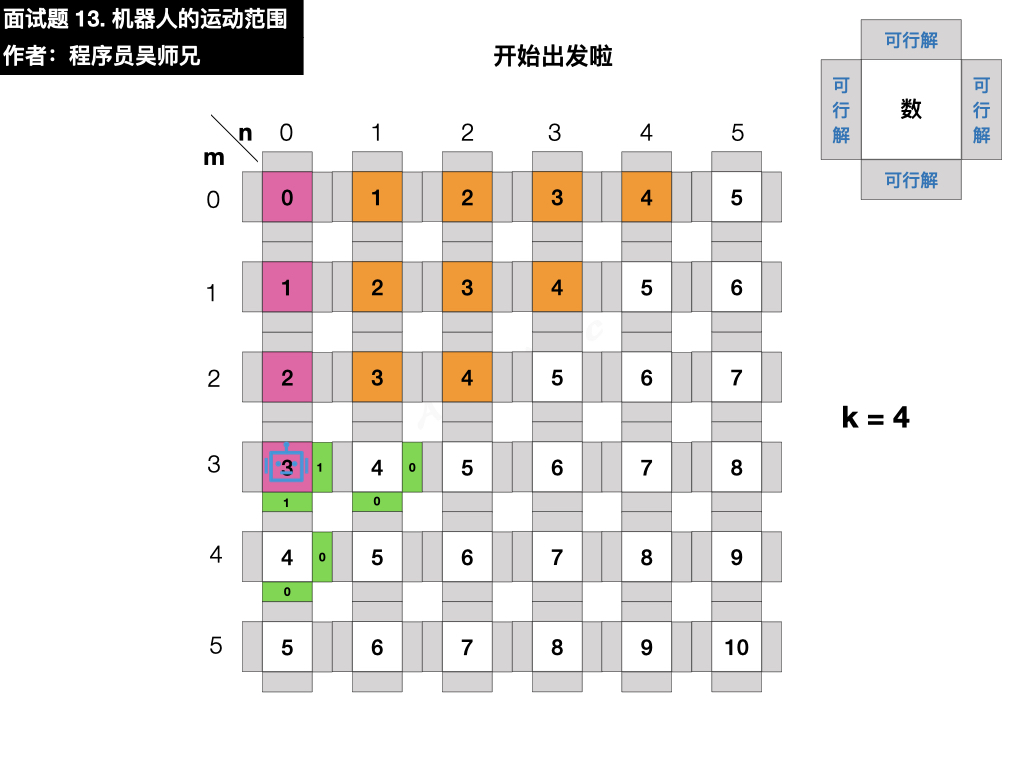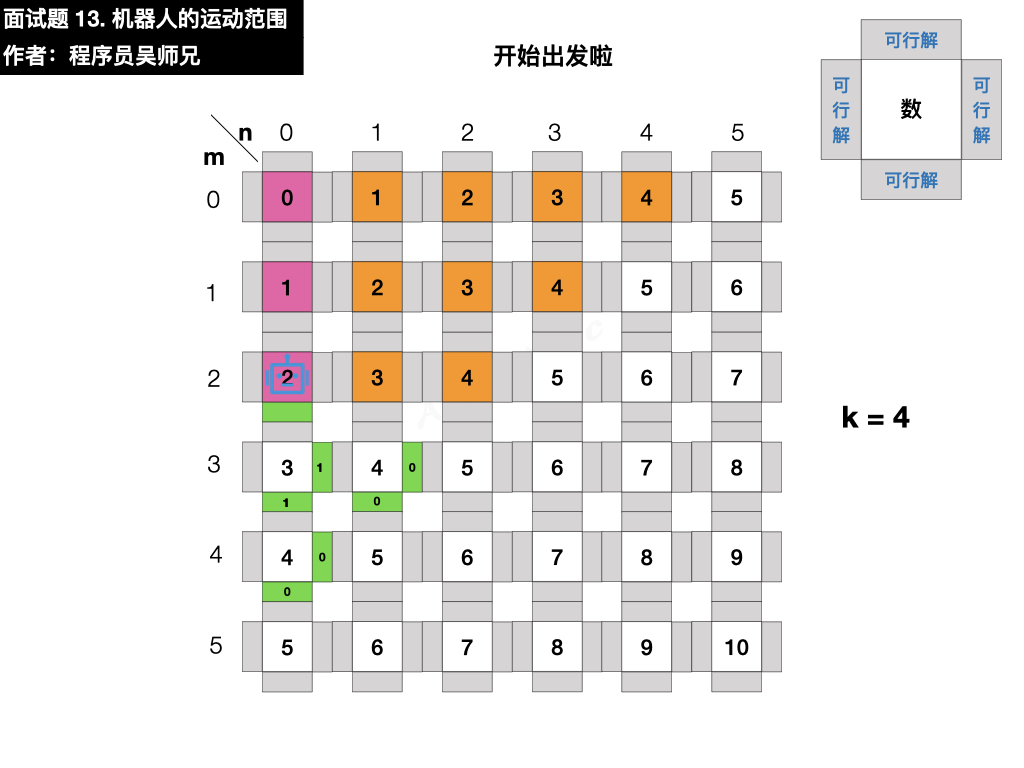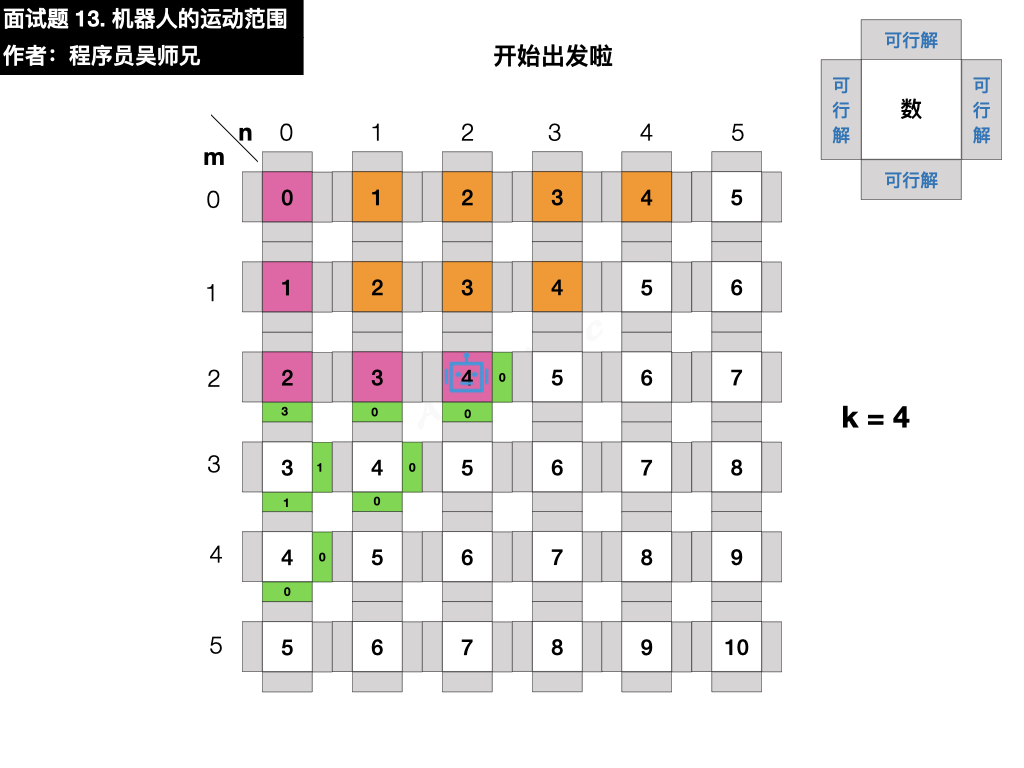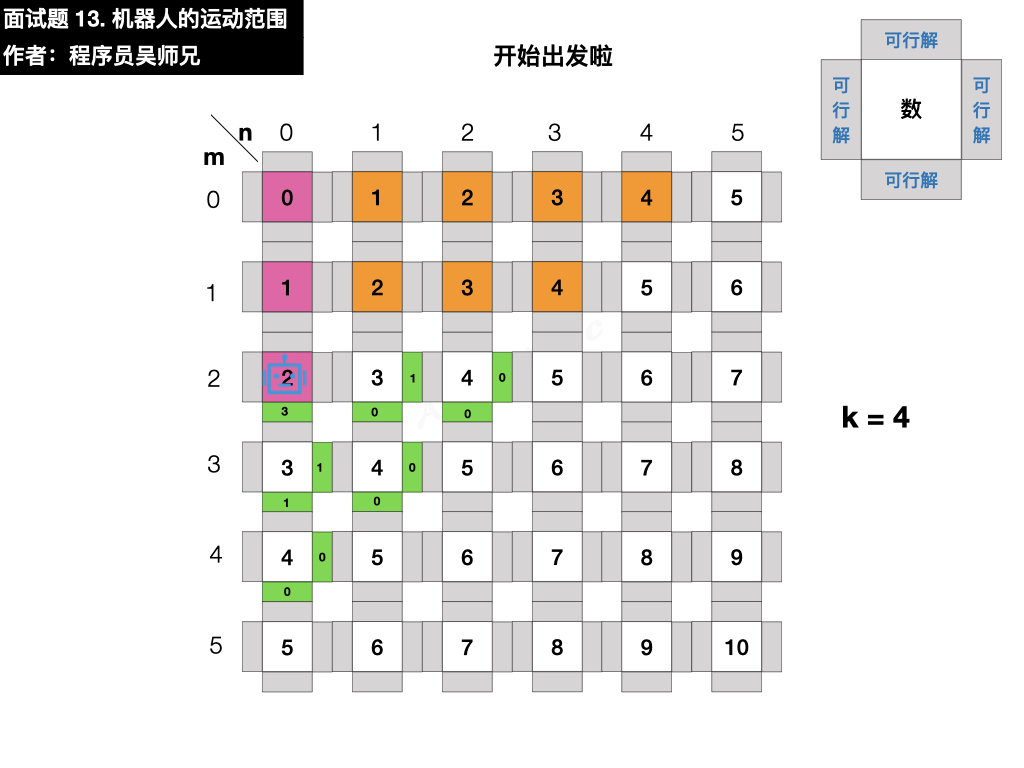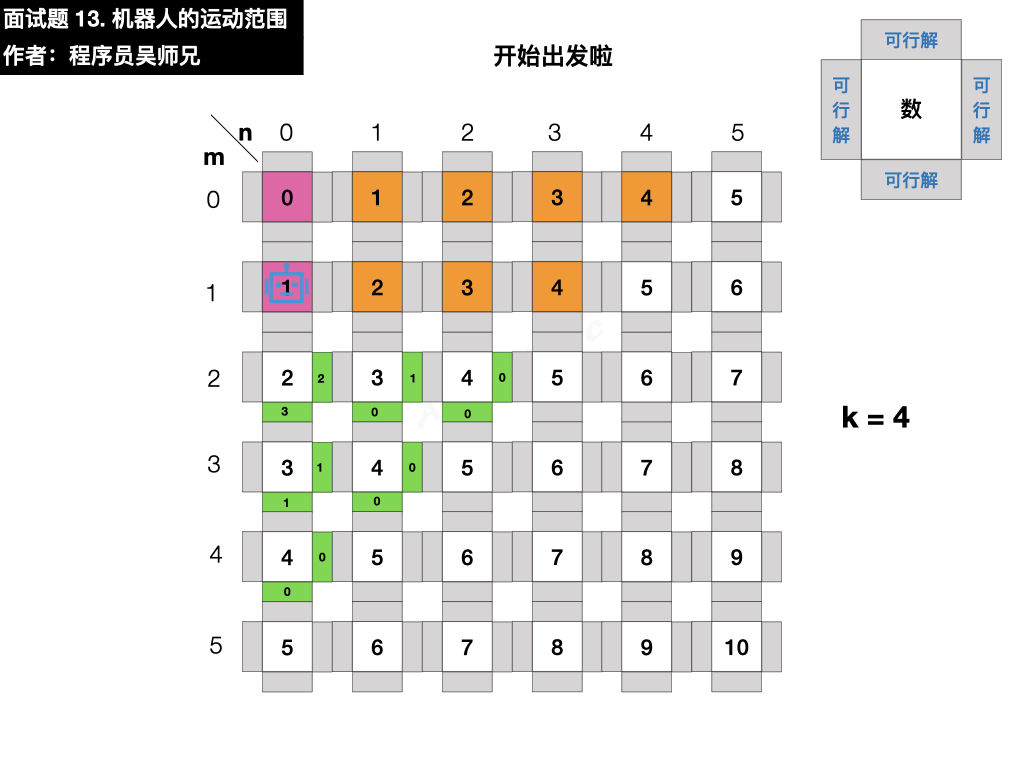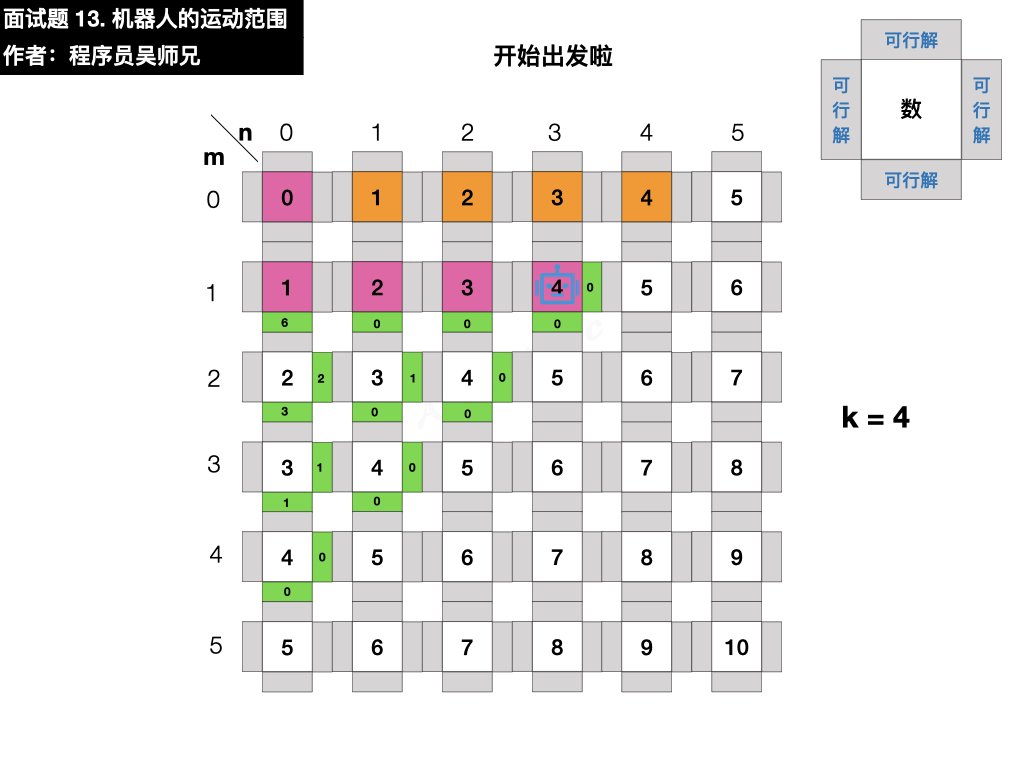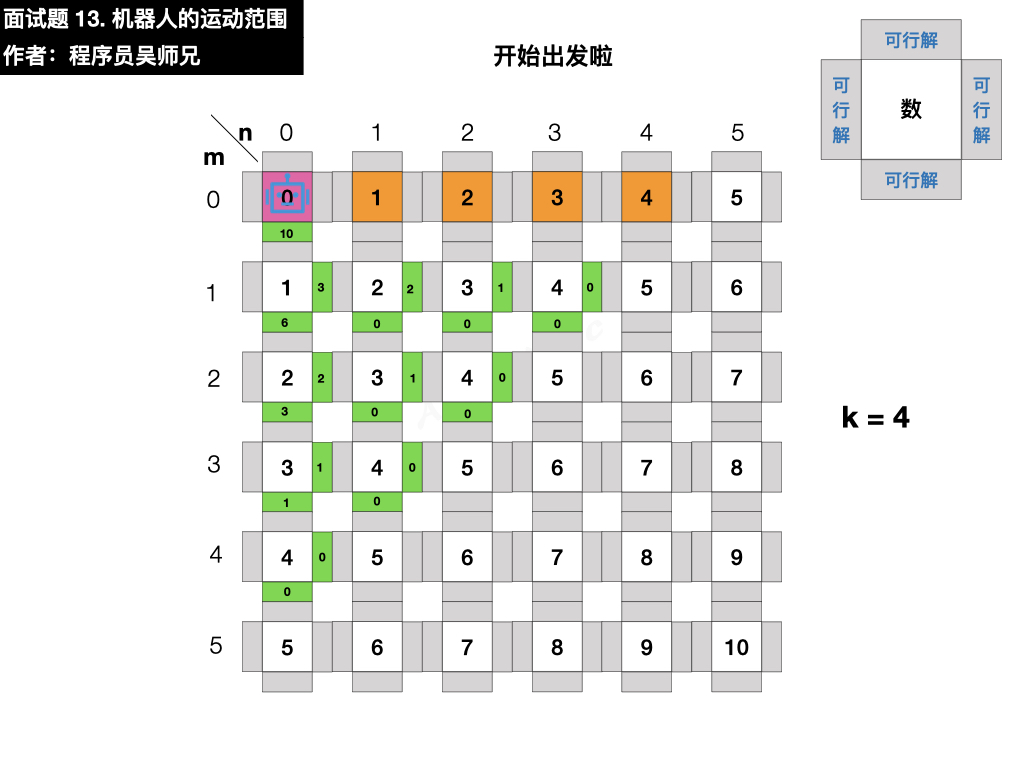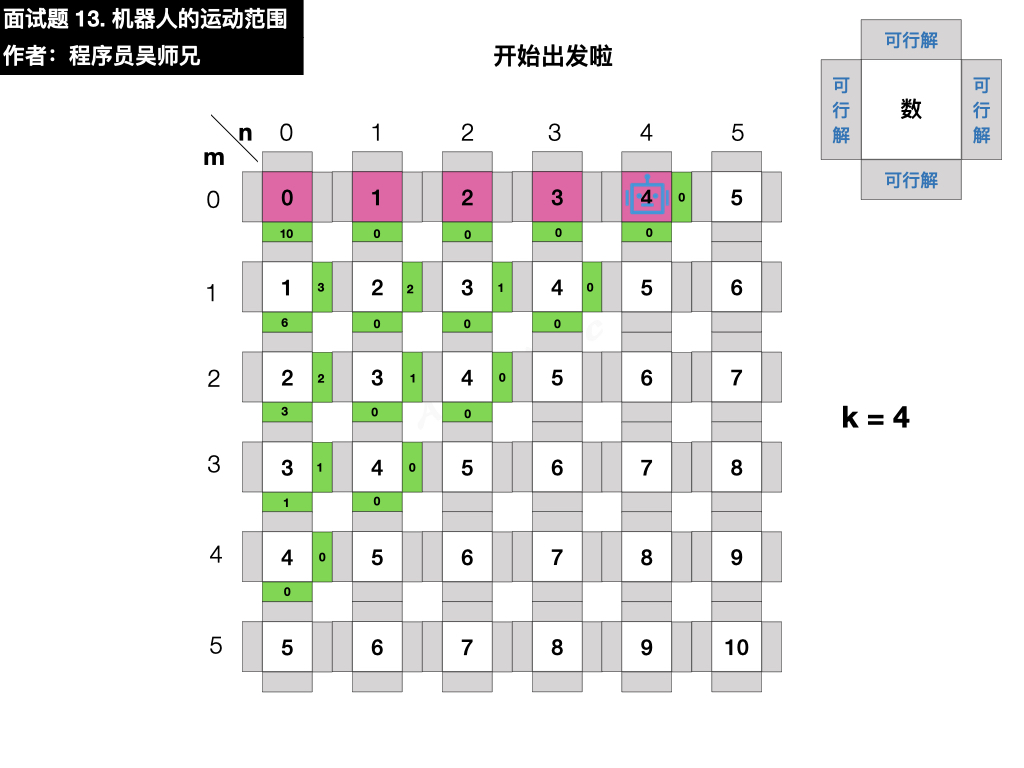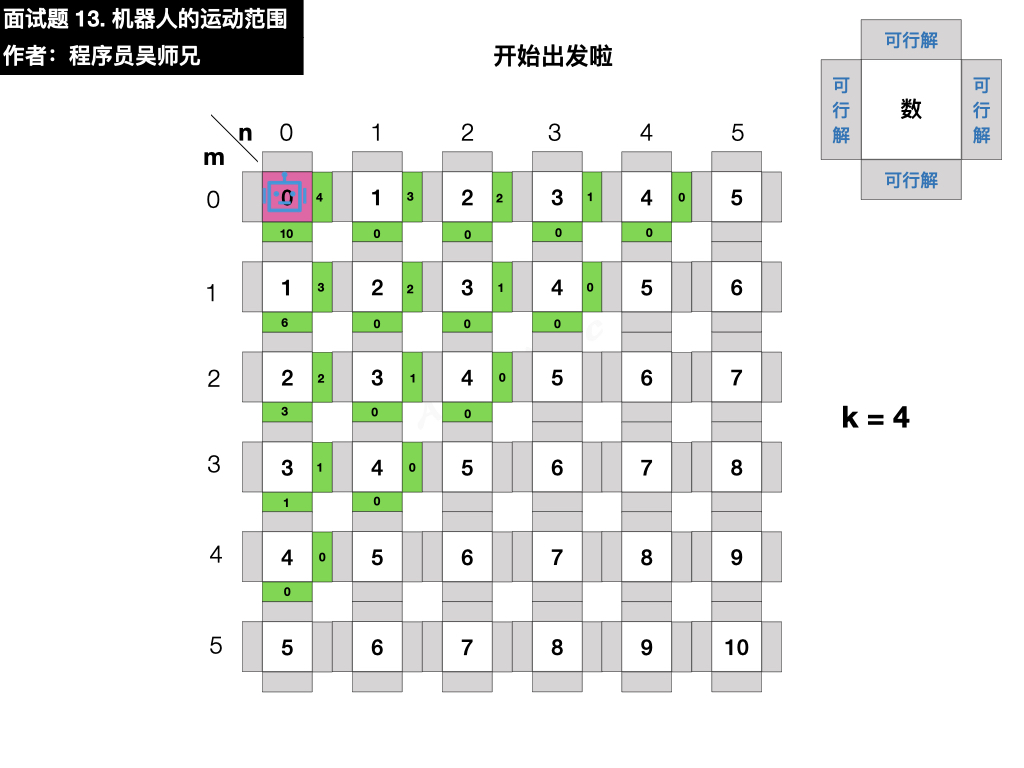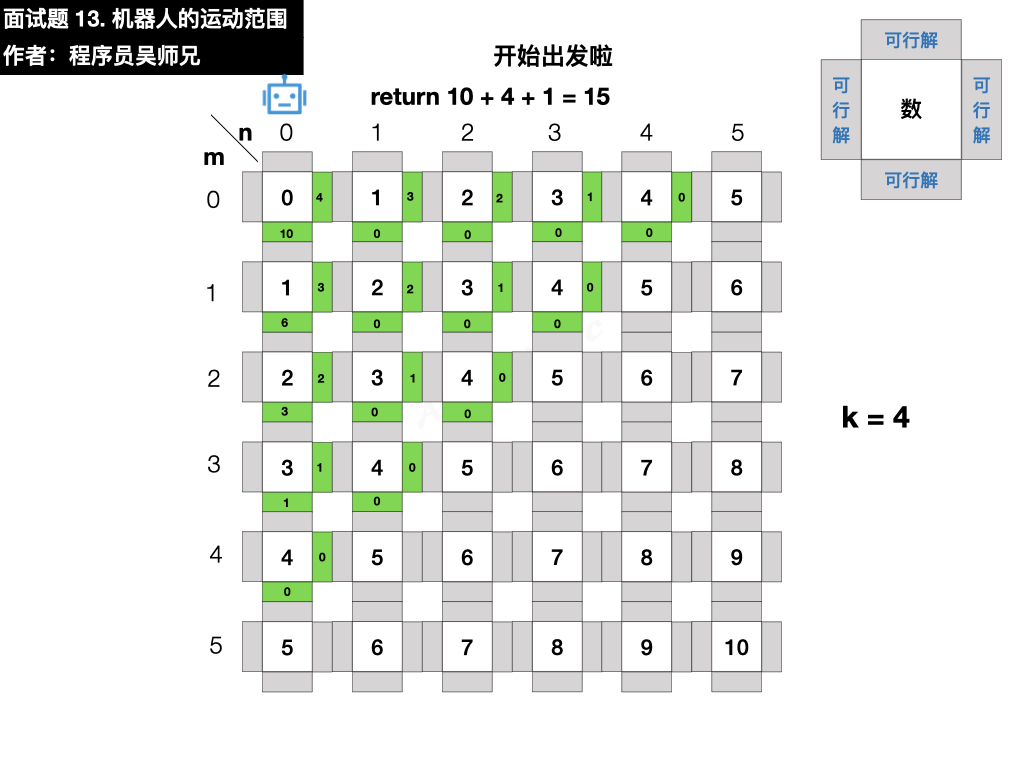#### 3、匹配

• 递归参数： 当前元素在矩阵中的行列索引 i 和 j ，两者的数位和 sumi, sumj 。
• 终止条件：
• ① 行列索引越界
• ② 数位和超出目标值 k ，即不满足行坐标和列坐标的数位之和小于 k 的格子
• ③ 已经被访问过的重复格子
• 递推工作：

• 标记当前单元格 ：将索引 (i, j) 存入临时变量 visited 中，代表此单元格已被访问过。
• 搜索下一单元格： 计算当前元素的 下、右 两个方向元素的数位和，并开启下层递归 。
• 回溯返回值： 返回 1 + 右方搜索的可达解总数 + 下方搜索的可达解总数，代表从本单元格递归搜索的可达解总数。

#### 4、边界

• 超出边界的格子
• 已经被访问过的重复格子
• 不满足行坐标和列坐标的数位之和小于 k 的格子

### 三、动画描述

• 普通用户特权：5积分
• 会员用户特权：免费
• 算法训练营永久会员用户特权：免费推荐

### 四、图片描述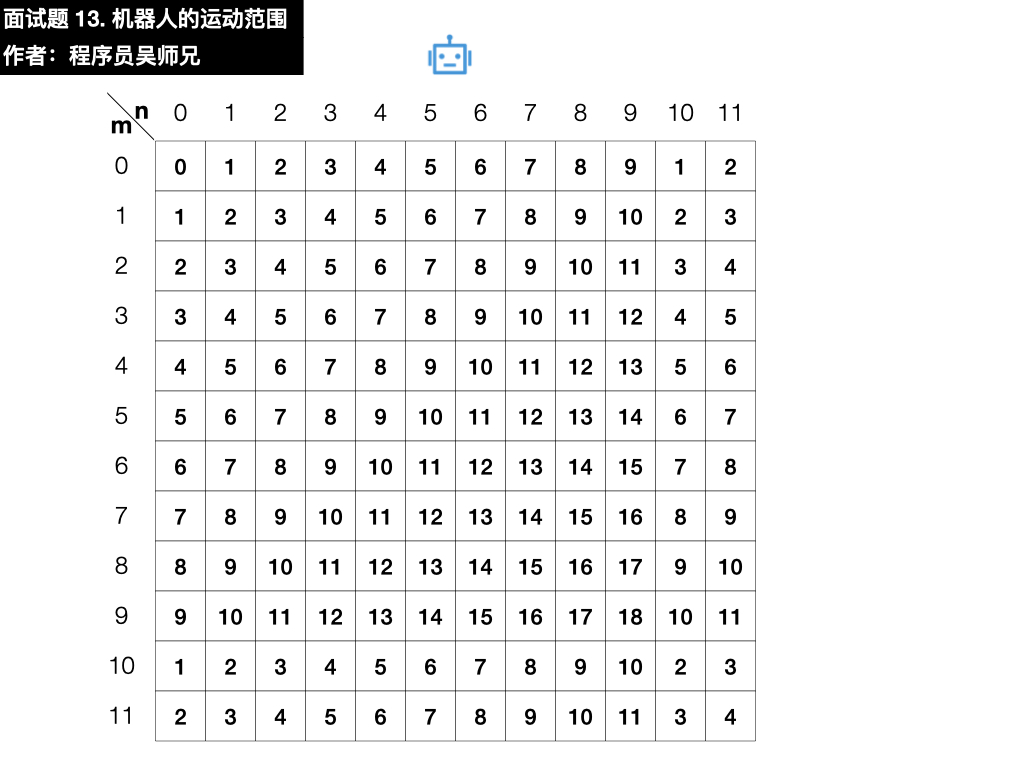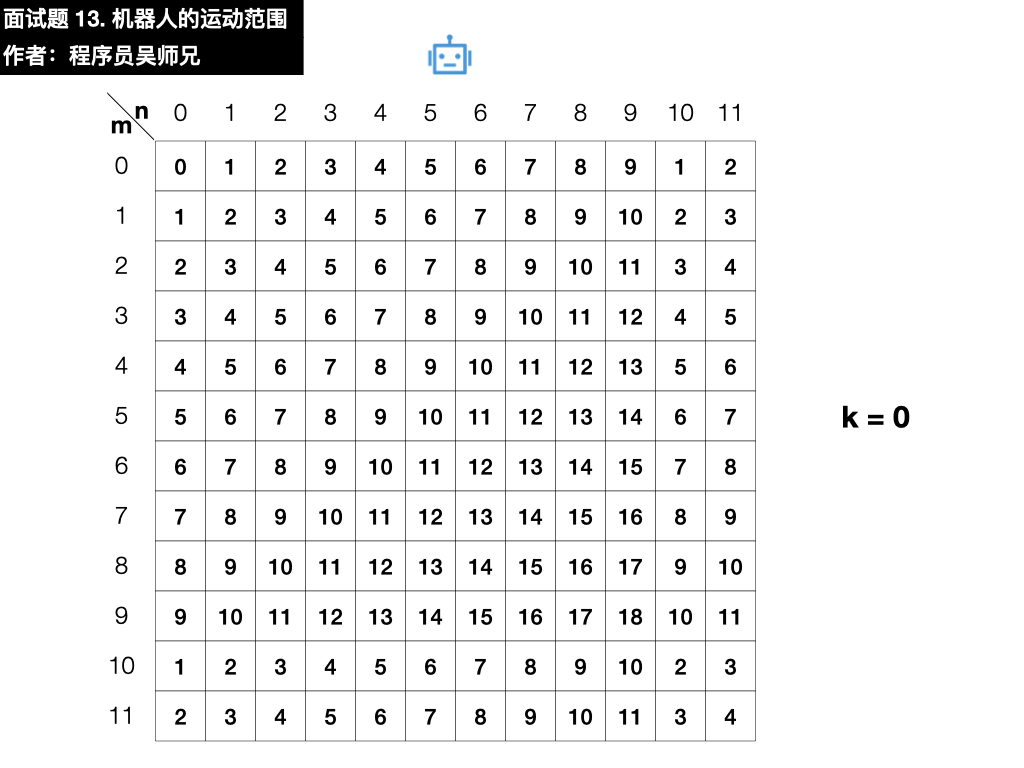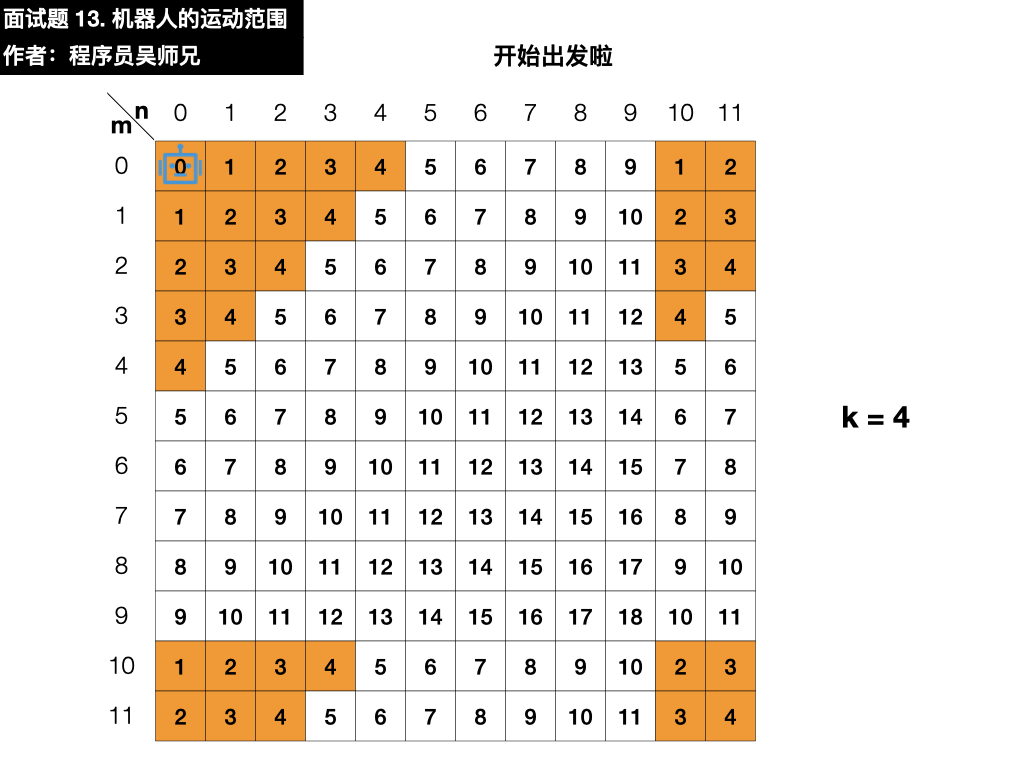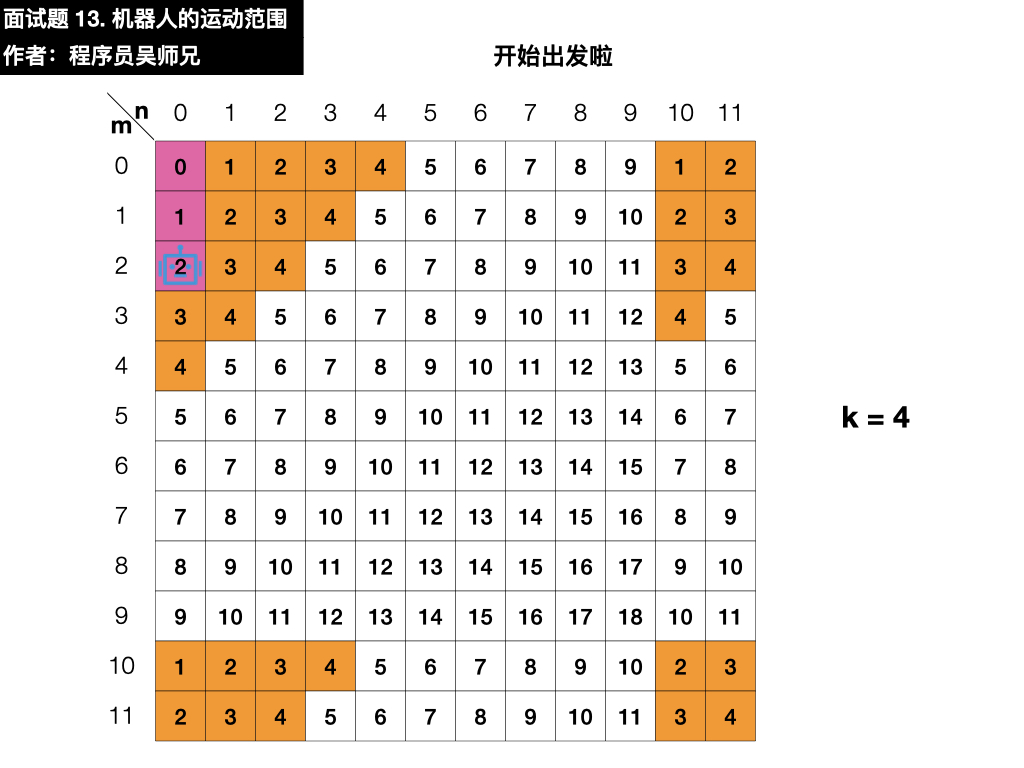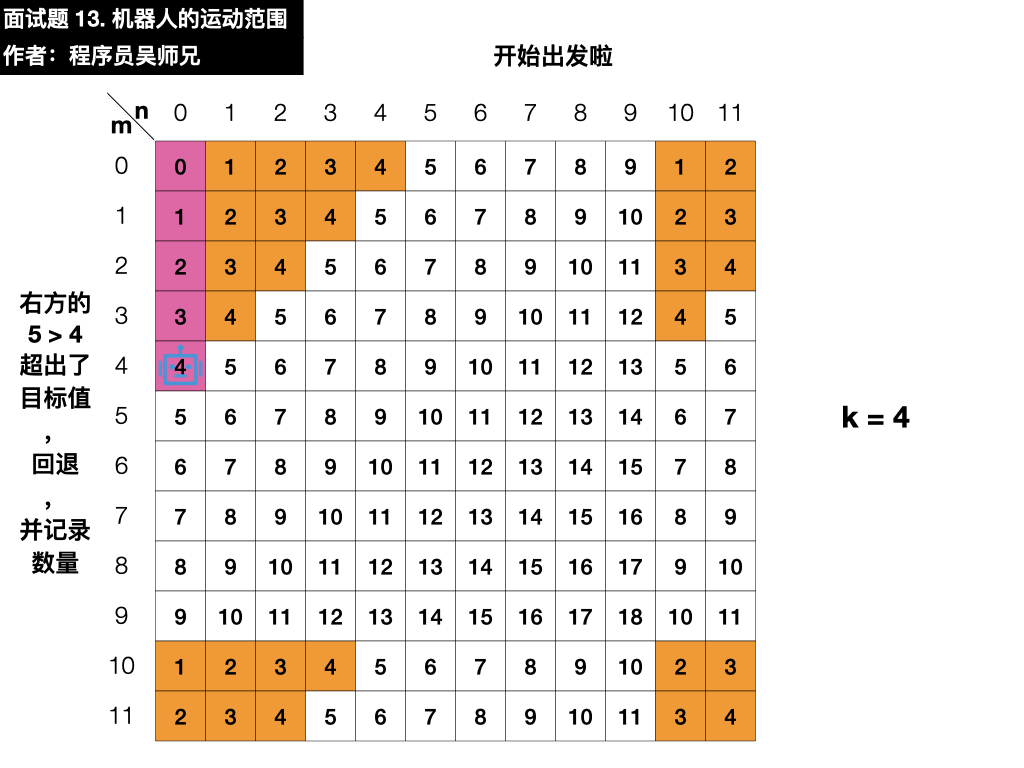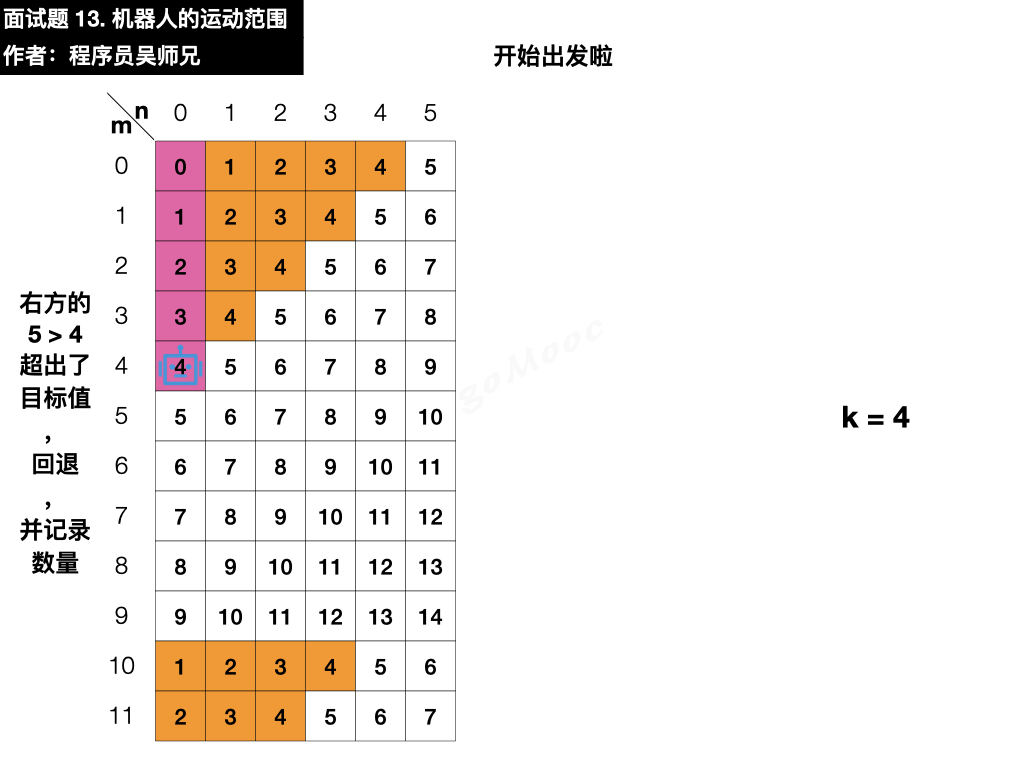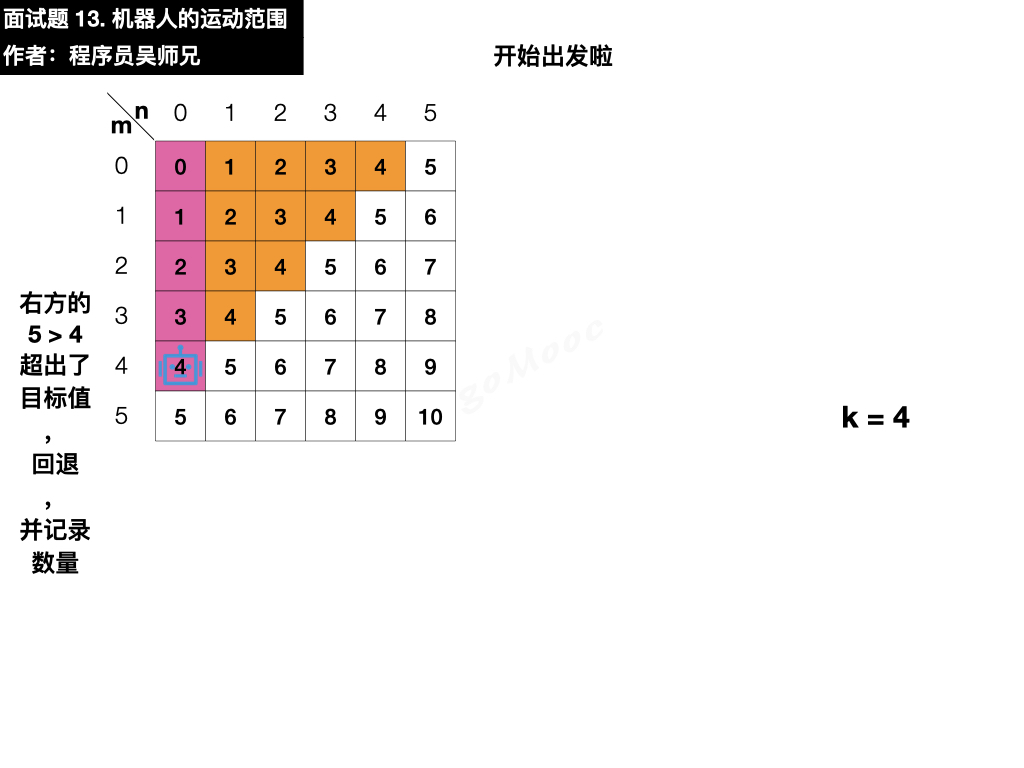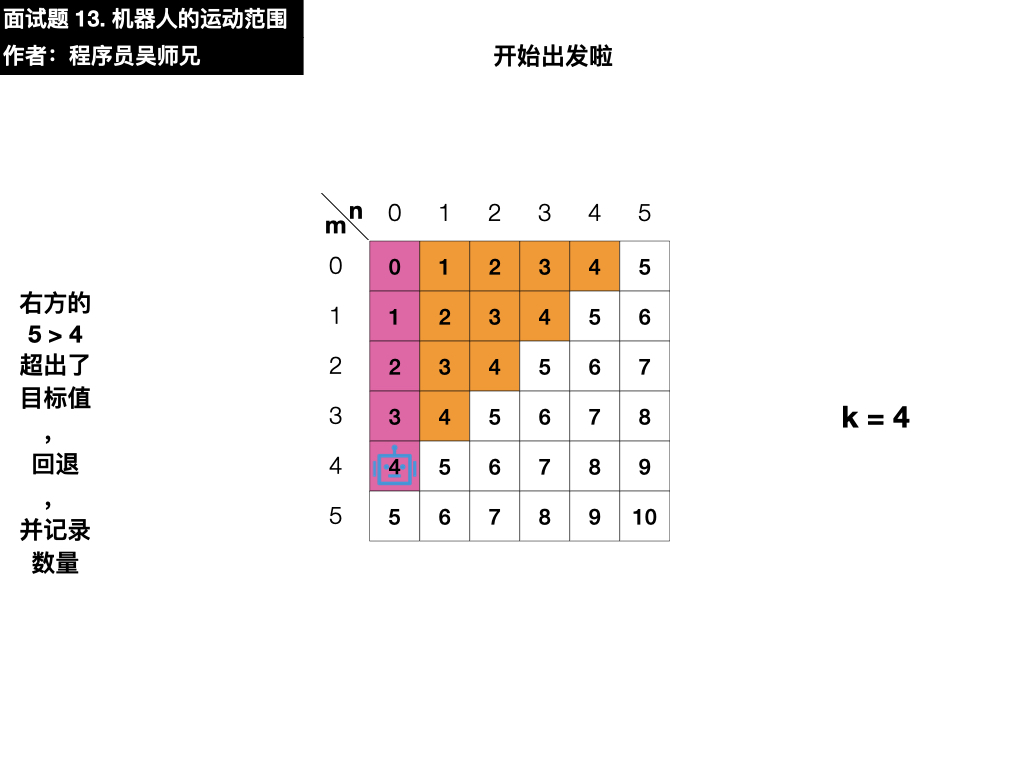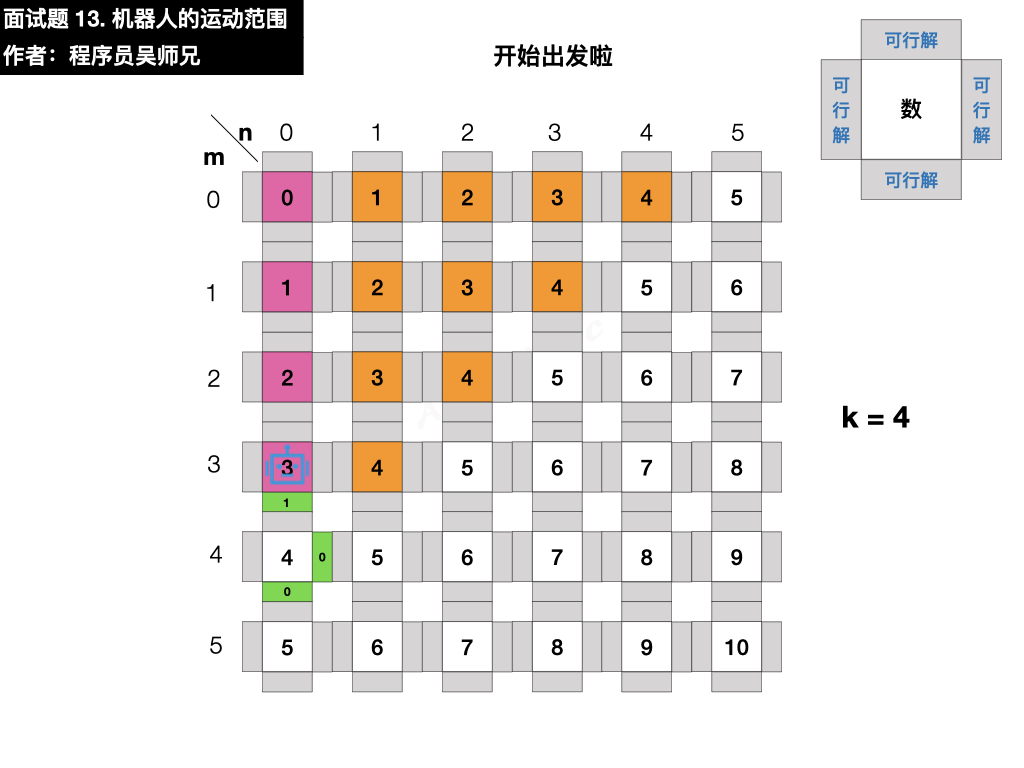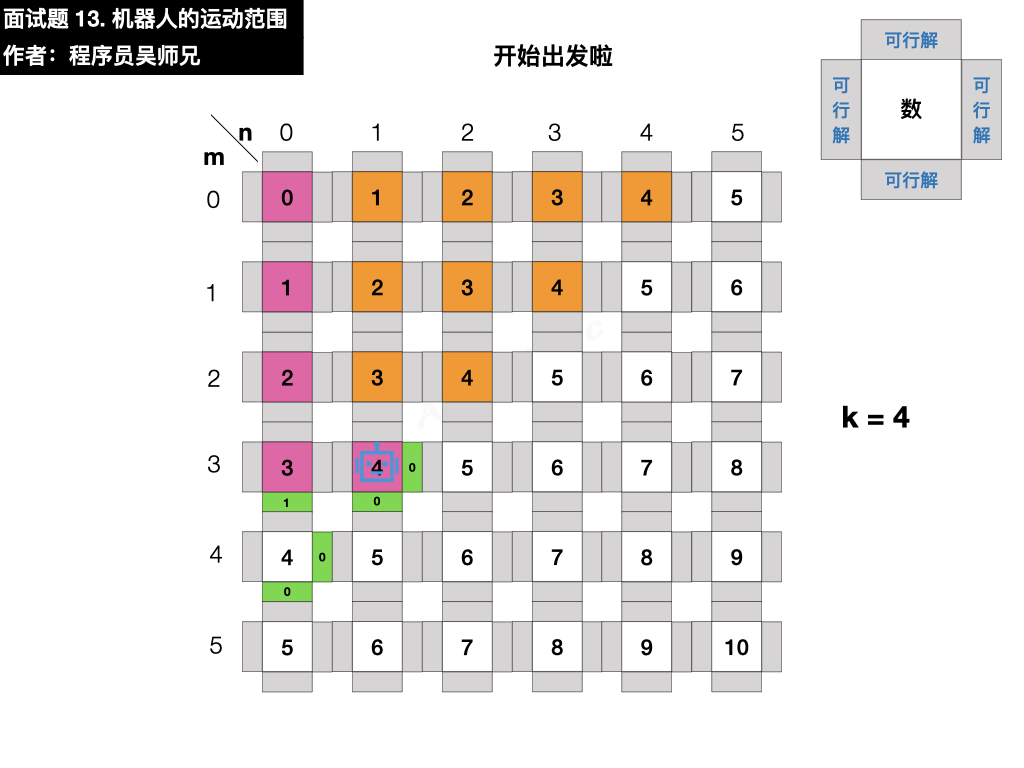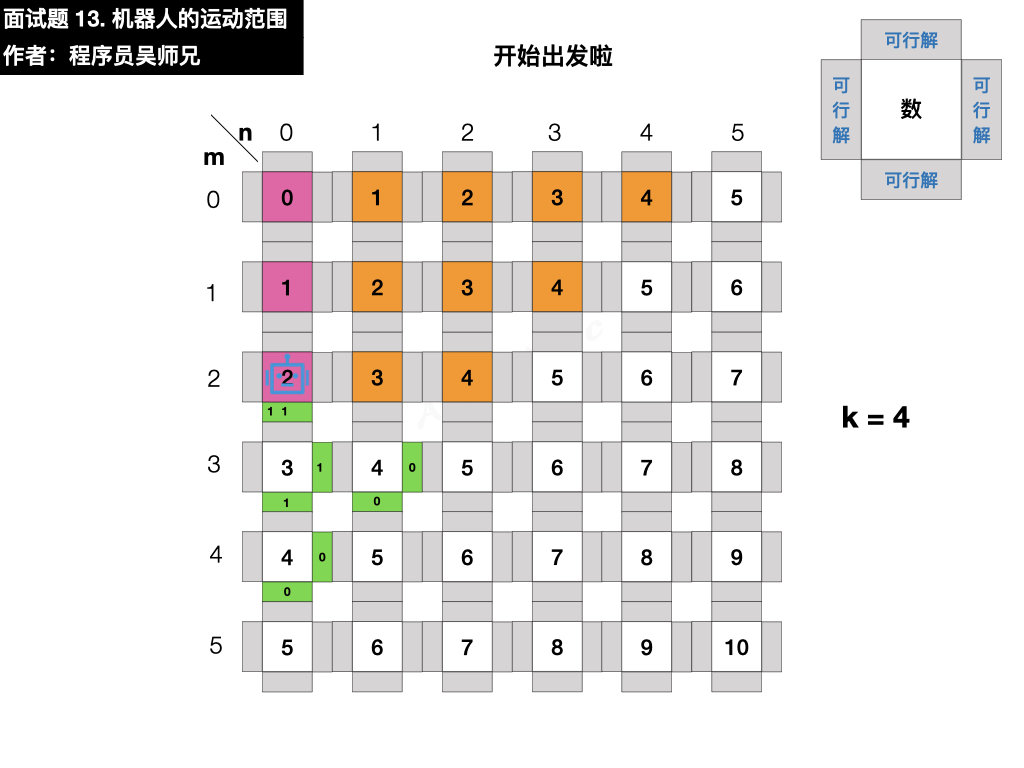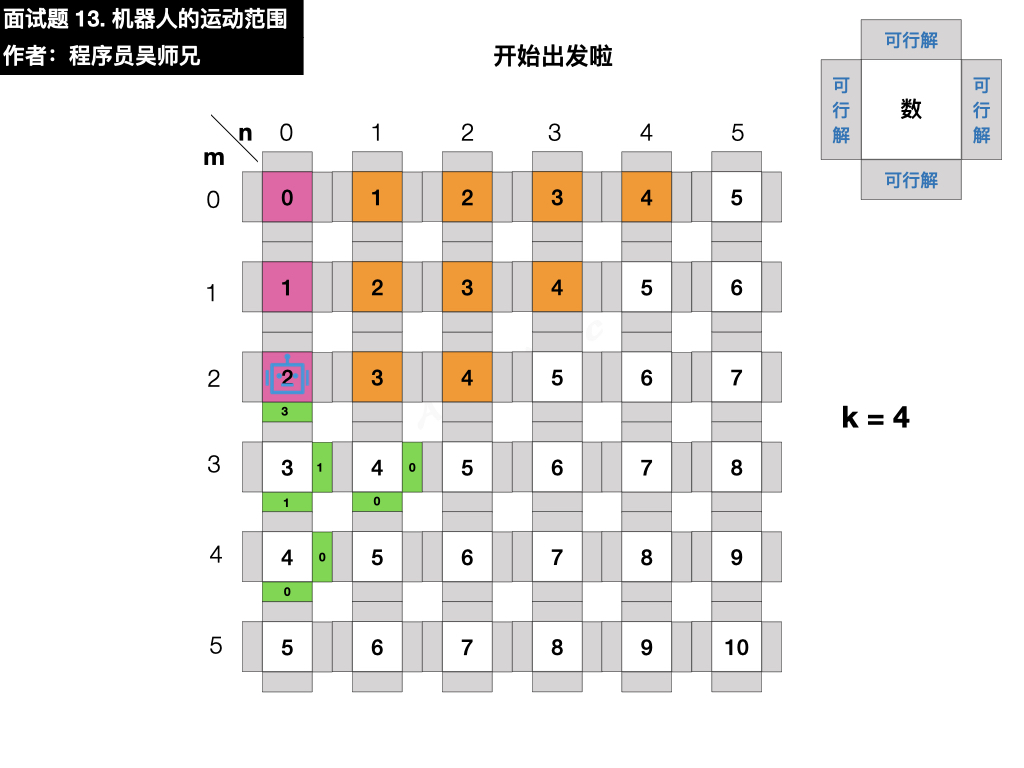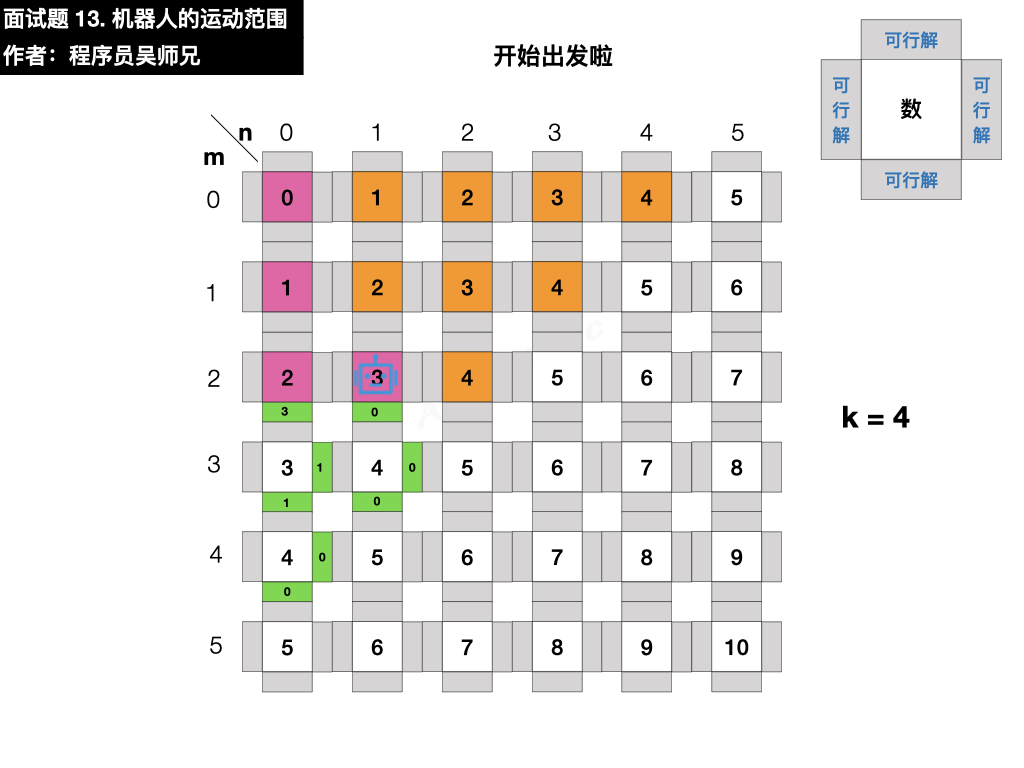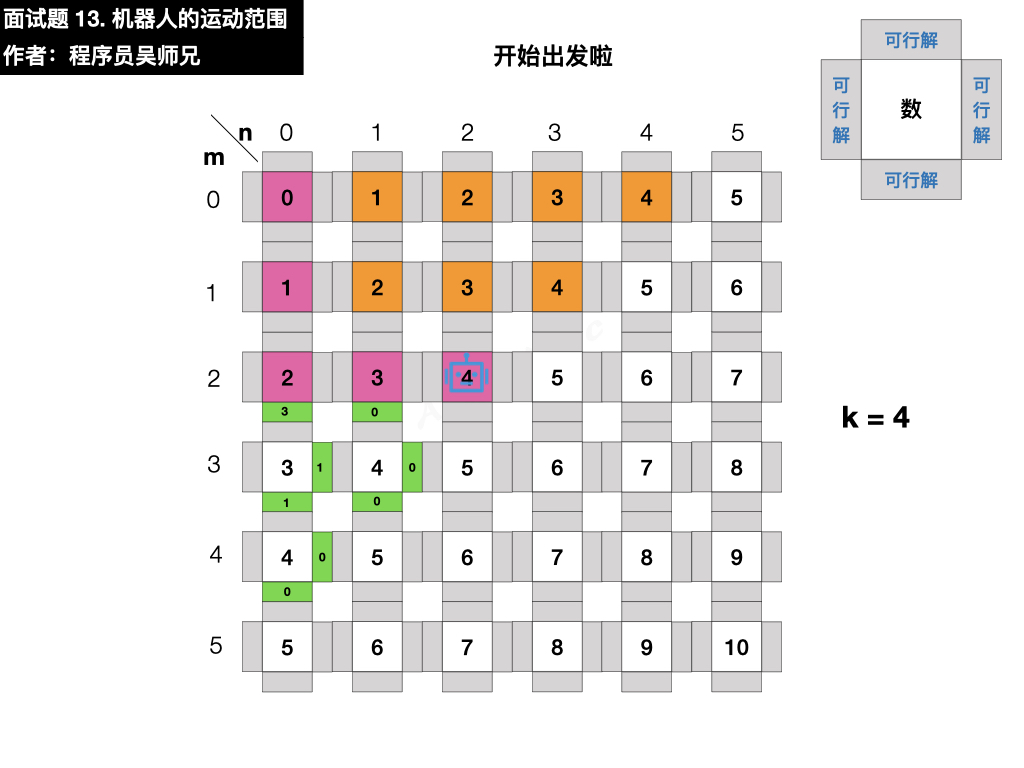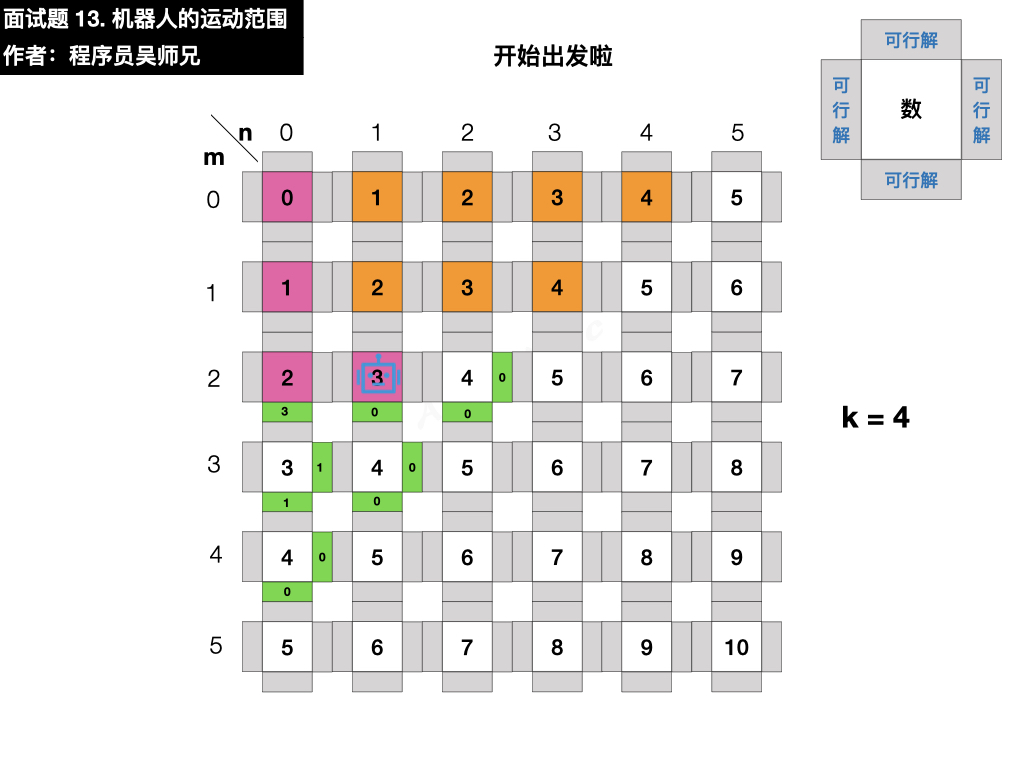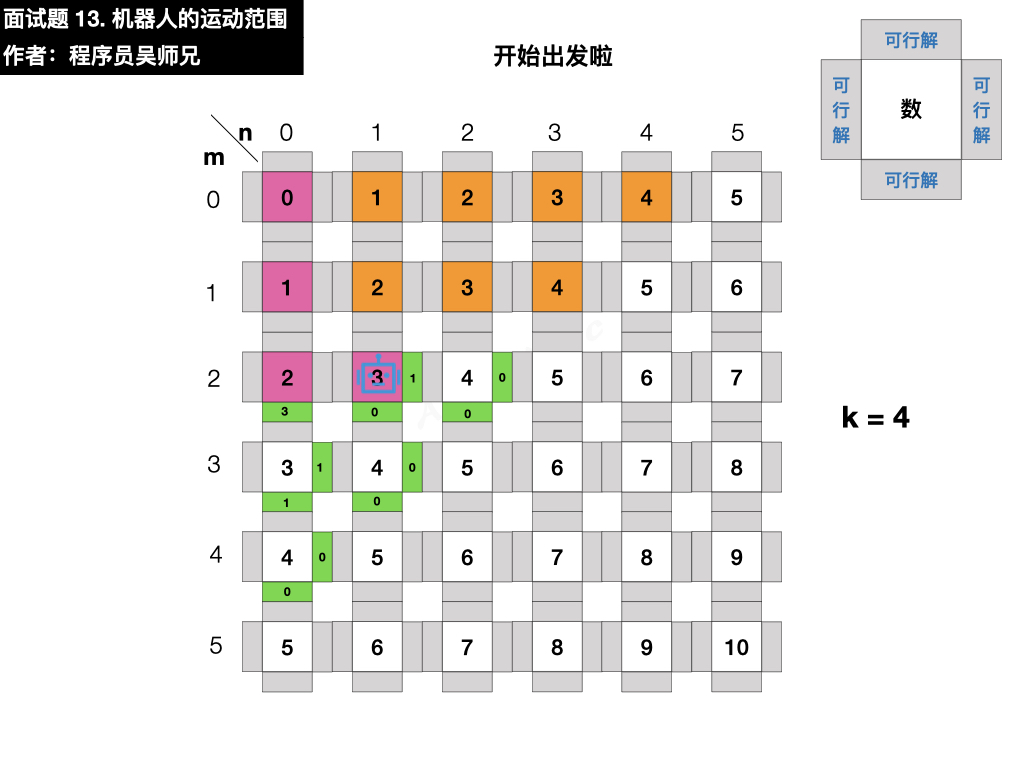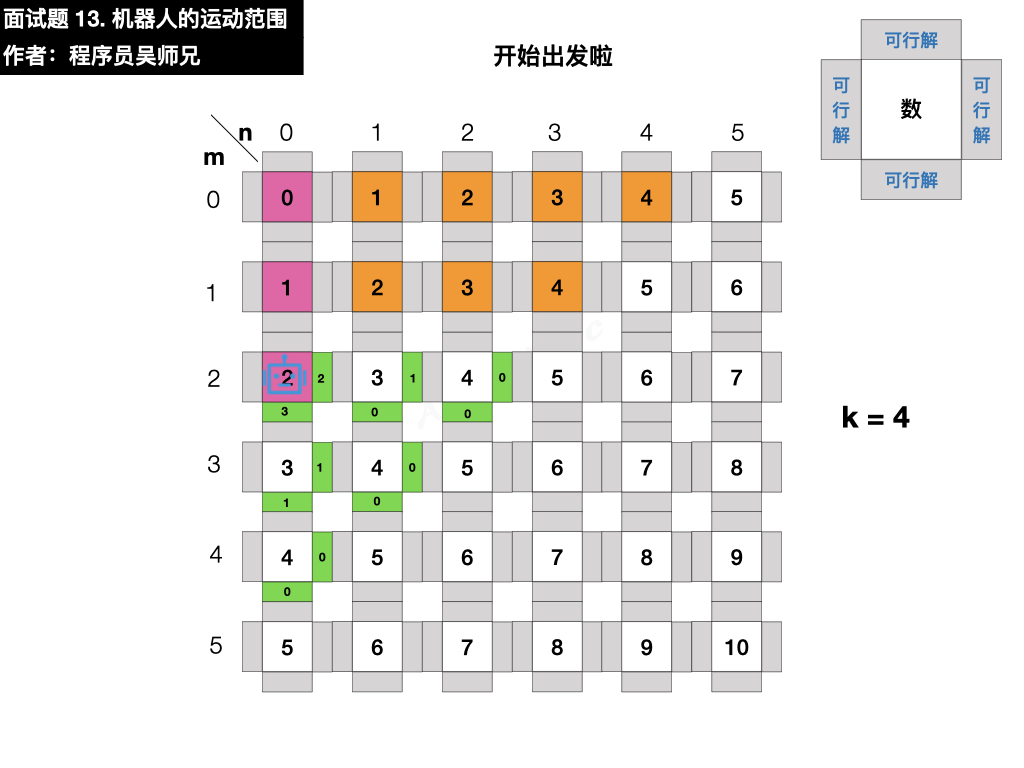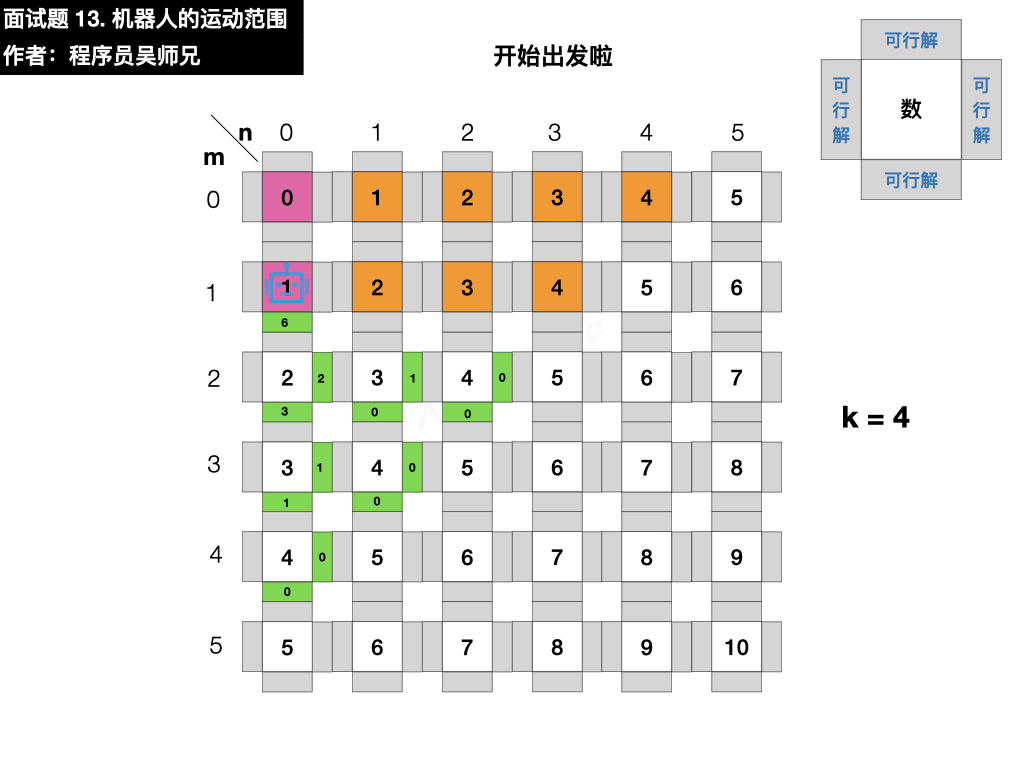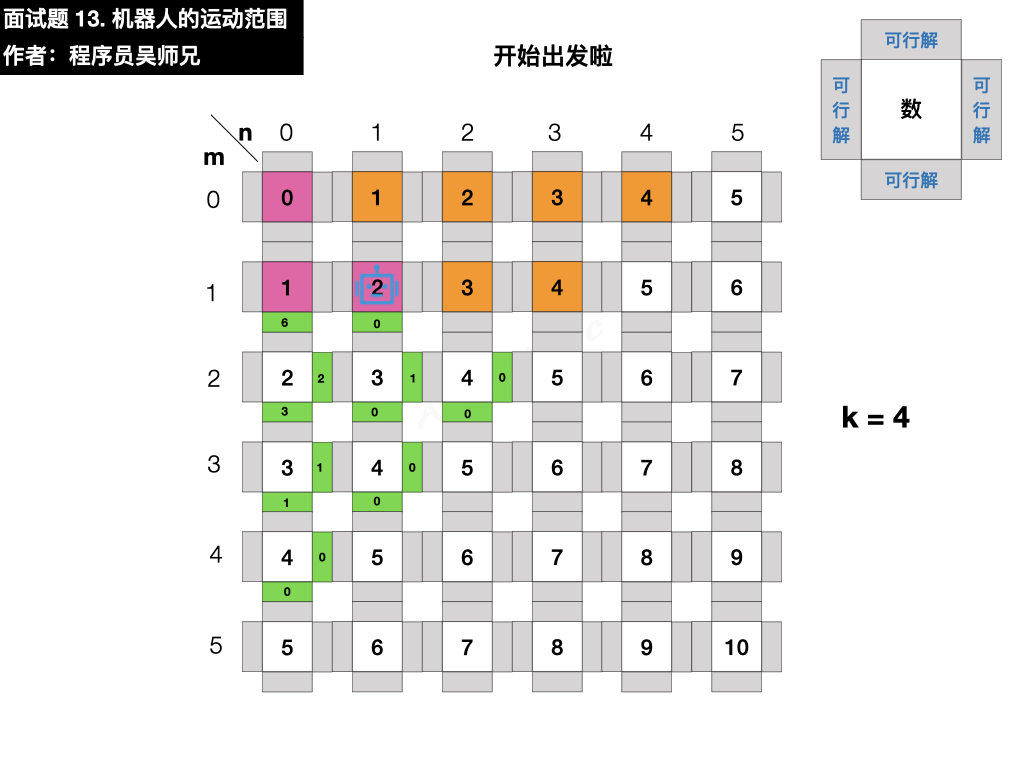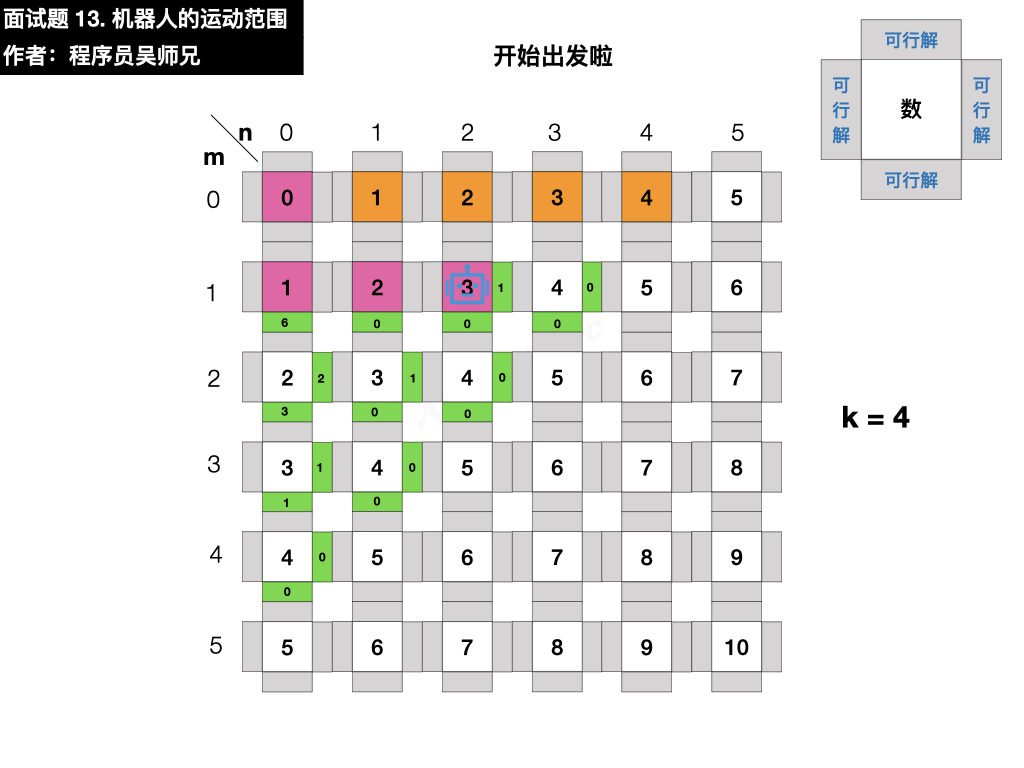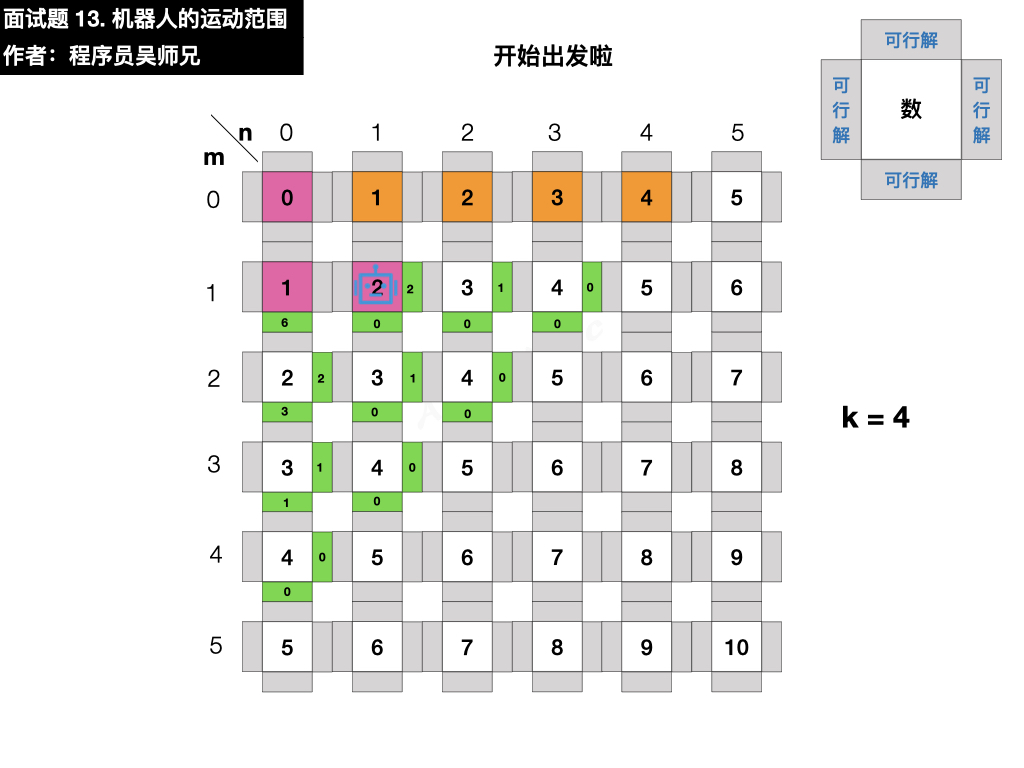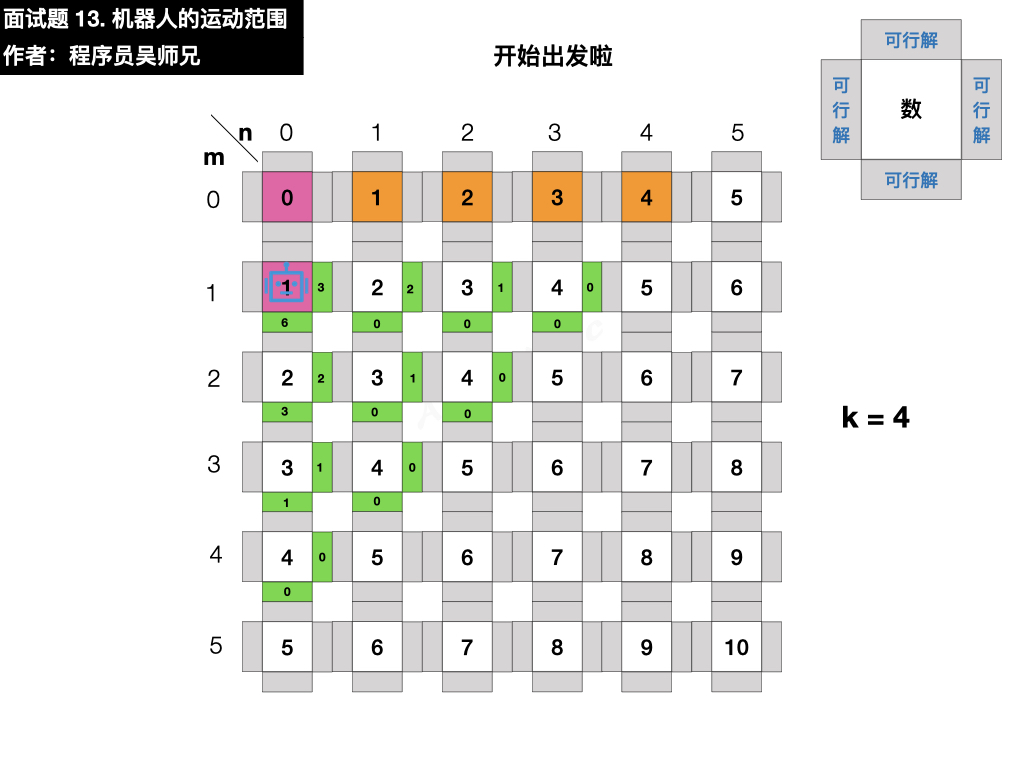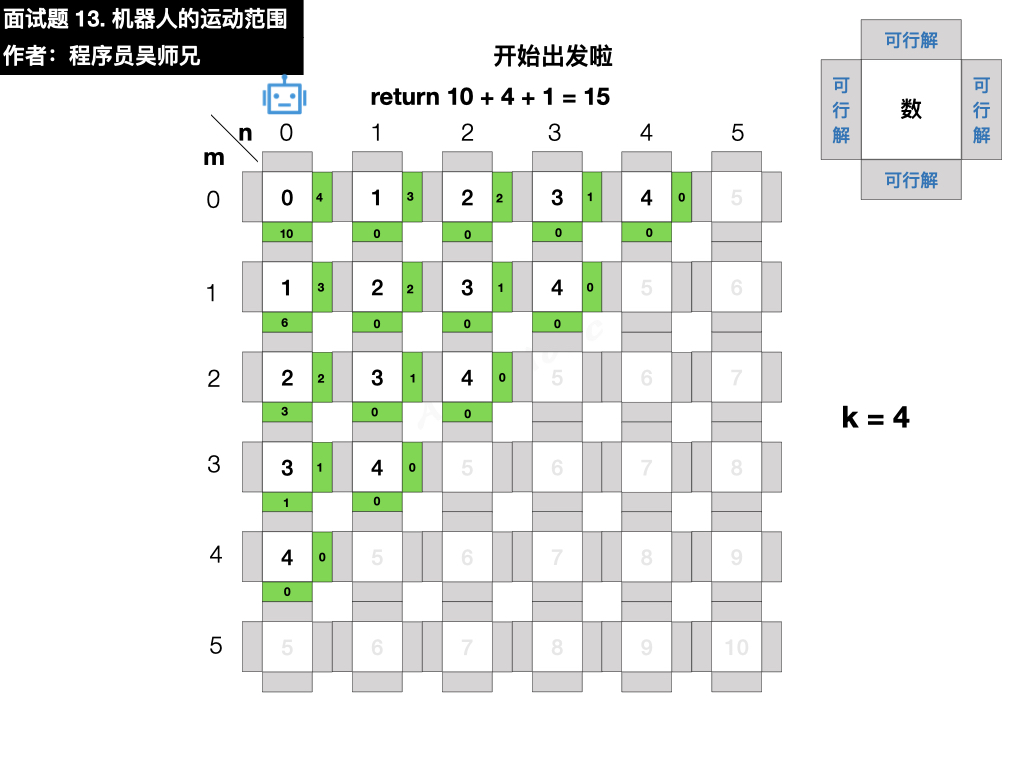### 五、参考代码

// 登录 AlgoMooc 官网获取更多算法图解
// https://www.algomooc.com
class Solution {
public int movingCount(int m, int n, int k) {
// 使用临时变量 visited 记录格子是否被访问过
boolean[][] visited = new boolean[m][n];
// 开始深度优先遍历
return dfs(0, 0, m, n, k, visited);
}

public int dfs(int i, int j, int m, int n, int k, boolean[][] visited) {
// 行列索引越界
if( i >= m || j >= n ) return 0;

// 数位和超出目标值 k ，即不满足行坐标和列坐标的数位之和小于 k 的格子
if( k < sum( i , j ) ) return 0;

// 已经被访问过的重复格子
if ( visited[i][j] ) return 0;

//机器人进入了一个新格子，标注这个格子被访问过
visited[i][j] = true;

//沿着当前格子的右边和下边继续访问
return 1 + dfs( i + 1, j , m ,  n , k , visited ) + dfs( i , j + 1, m , n , k , visited);

}

//计算两个坐标数字的和
private int sum(int i, int j) {
int sum = 0;

while (i != 0) {
// 通过求余和取整的操作来计算
sum += i % 10;
i /= 10;
}
while (j != 0) {
sum += j % 10;
j /= 10;
}
return sum;
}
}


• 深度优先搜索
• 递归
• 数组
• 回溯

### 八、参考链接

• https://leetcode-cn.com/problems/ji-qi-ren-de-yun-dong-fan-wei-lcof/solution/mian-shi-ti-13-ji-qi-ren-de-yun-dong-fan-wei-dfs-b/
• https://leetcode-cn.com/problems/ji-qi-ren-de-yun-dong-fan-wei-lcof/
• https://www.algomooc.com/263.html